# RD Sharma Solutions for Class 11 Maths Chapter 3 Functions

## RD Sharma Solutions Class 11 Maths Chapter 3 – Free PDF Download

RD Sharma Solutions for Class 11 Maths Chapter 3 – Functions, we shall study about one of the most important concepts in Mathematics known as a Function. We shall also introduce the concept of a function as a correspondence between two sets, and function also has a relation from one set to the other set. BYJU’S tutor team has prepared solutions which help students attain good marks in Maths. From the exam point of view, the solutions are solved in a simple and structured manner where students can secure an excellent score by practicing the RD Sharma Class 11 Solutions for Maths textbook. Solutions that are provided here will help you in getting acquainted with a wide variety of questions and thus, develop problem-solving skills. PDFs can be downloaded easily from the links provided below.

Chapter 3- Functions contains four exercises and the RD Sharma Solutions present in this page provide solutions to the questions present in each exercise. Now, let us have a look at the concepts discussed in this chapter.

• Function as a special kind of relation.
• Function as a correspondence.
• Description of a function.
• Domain, co-domain and range of a function.
• Equal functions.
• Real functions.
• The domain of real functions.
• Range of real functions.
• Some standard real functions and their graphs.
• Operations on real functions.

## Download the Pdf of RD Sharma Solutions for Class 11 Maths Chapter 3 – Functions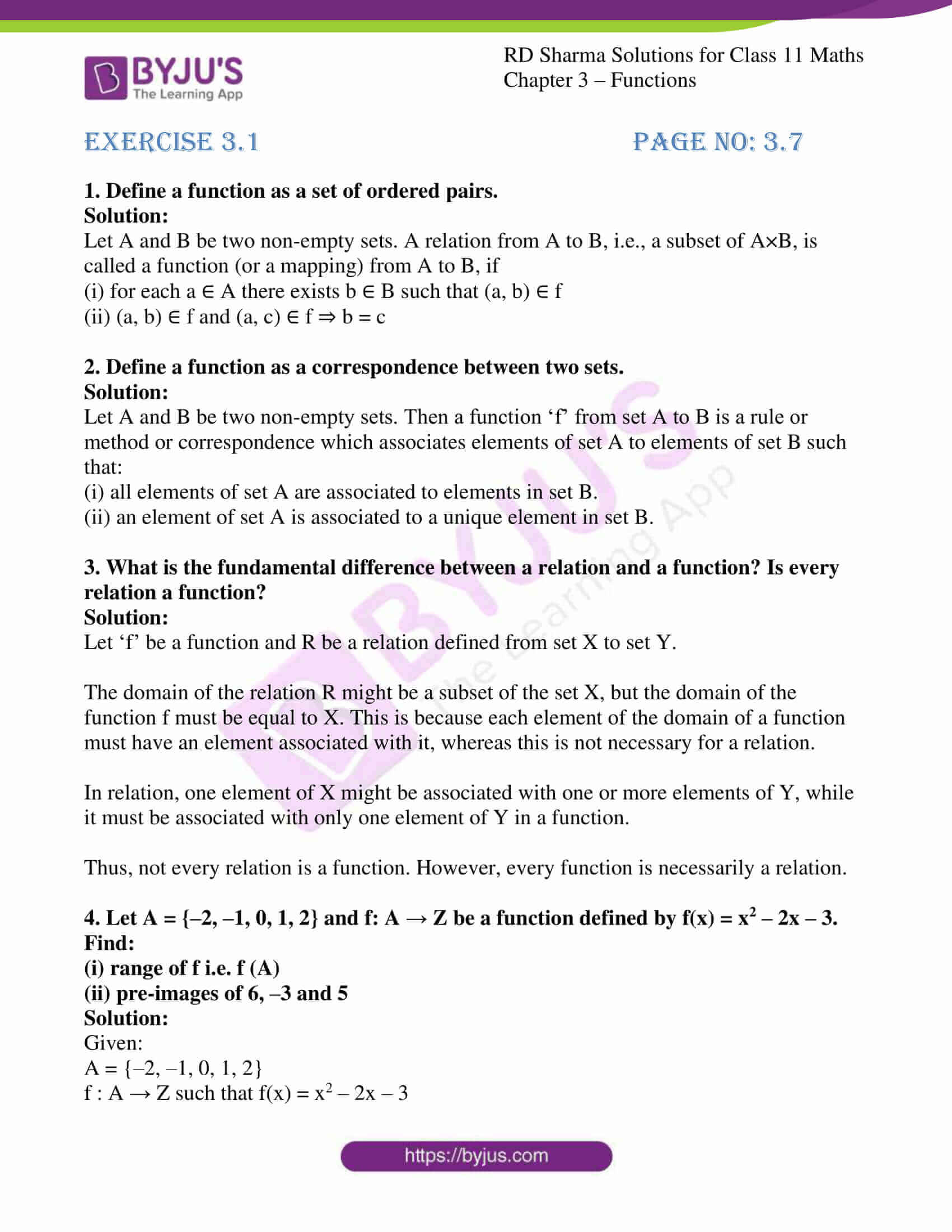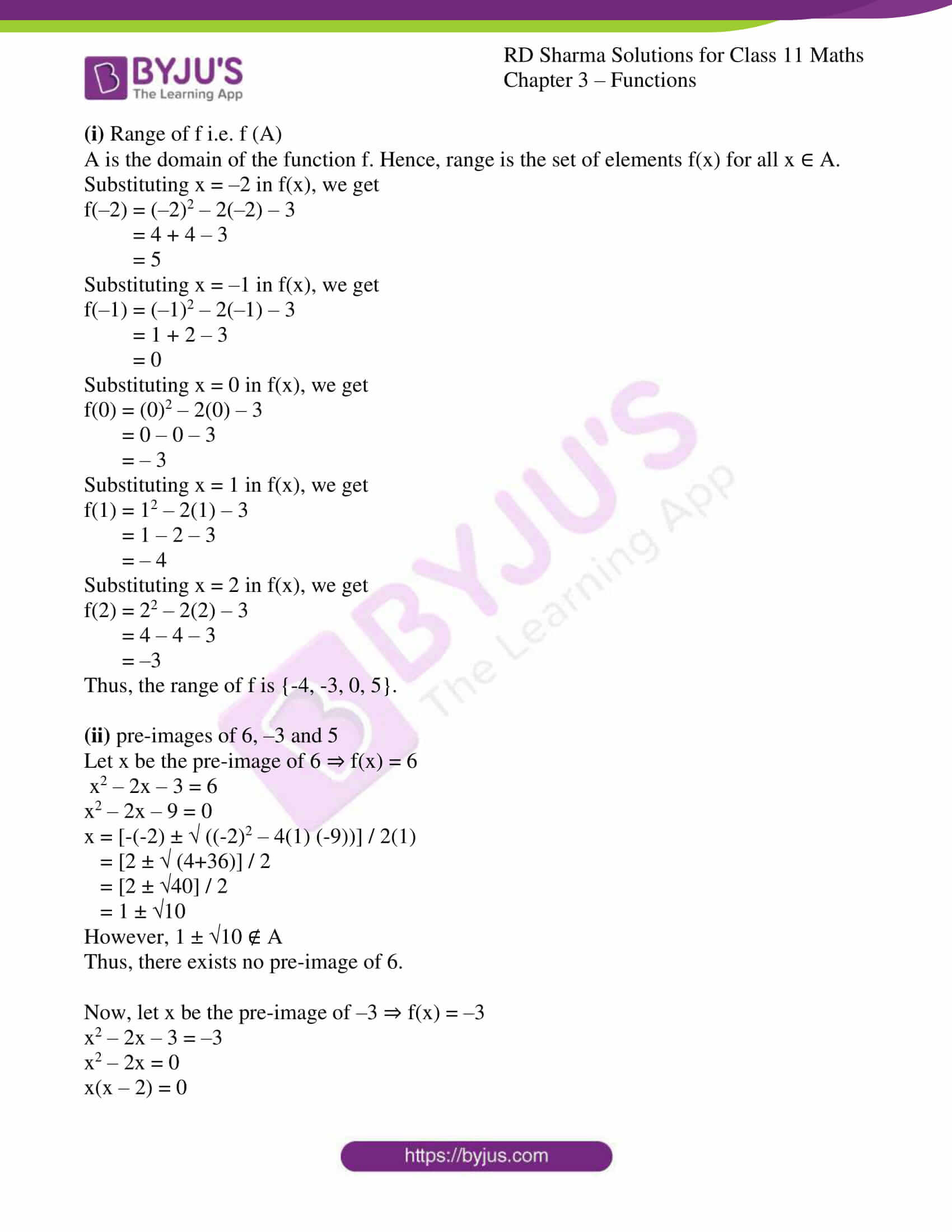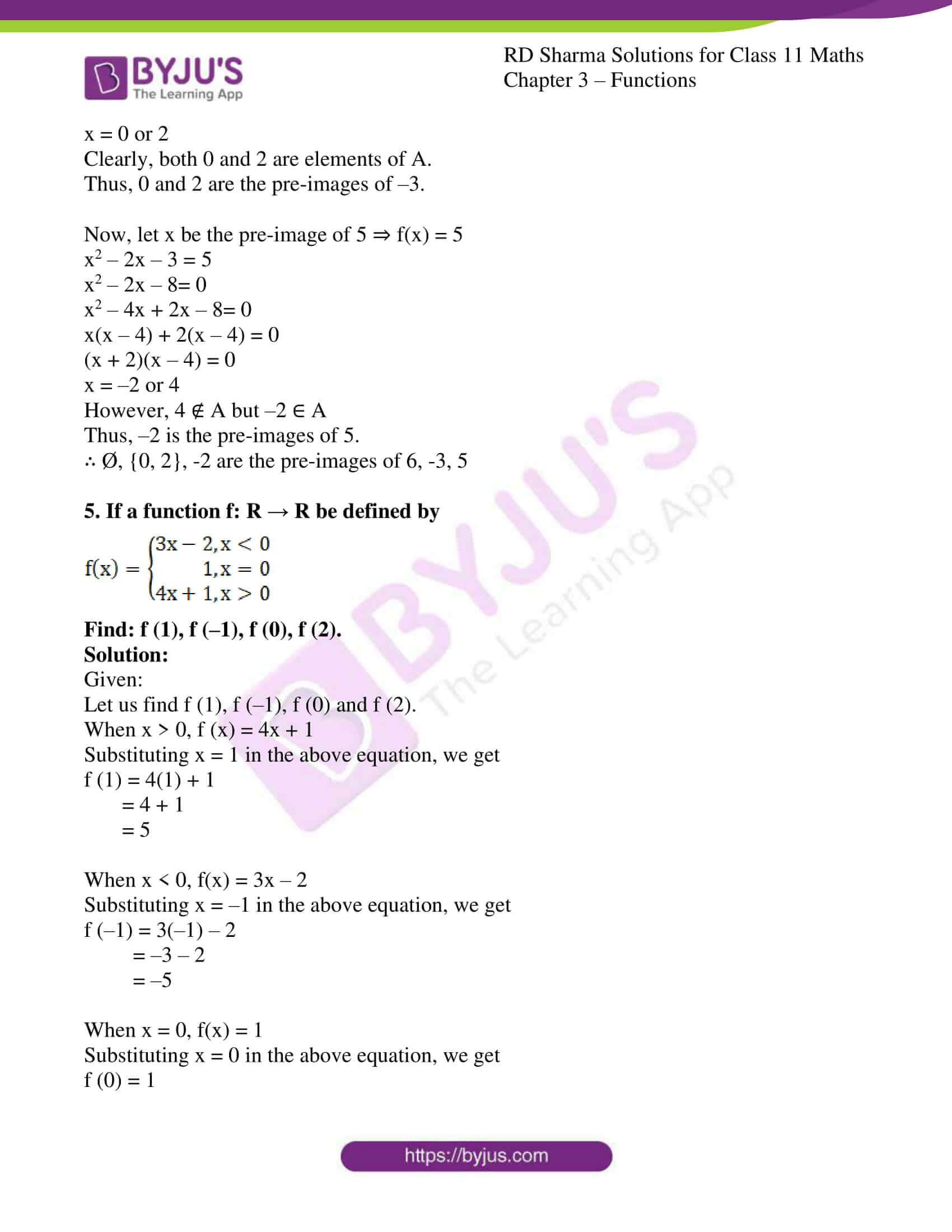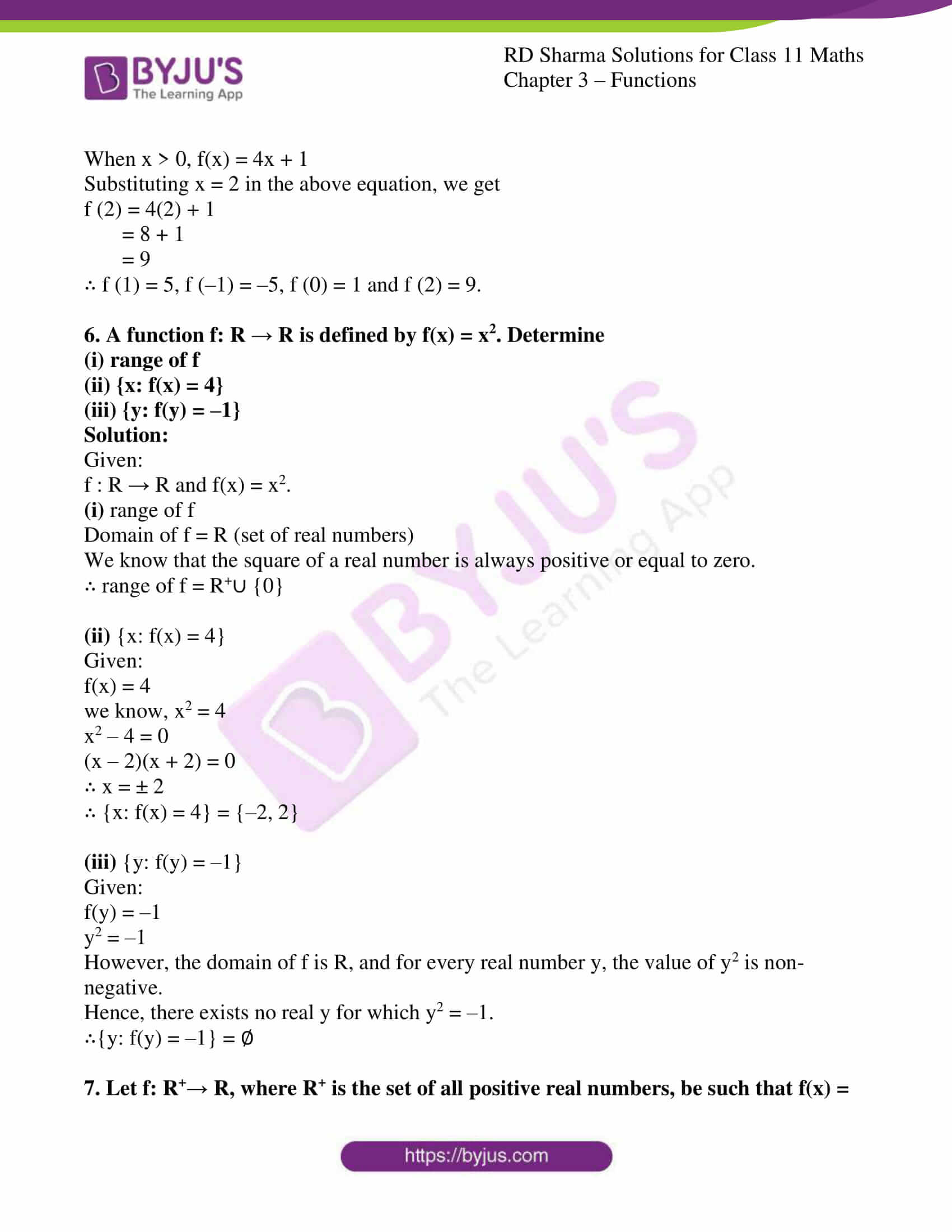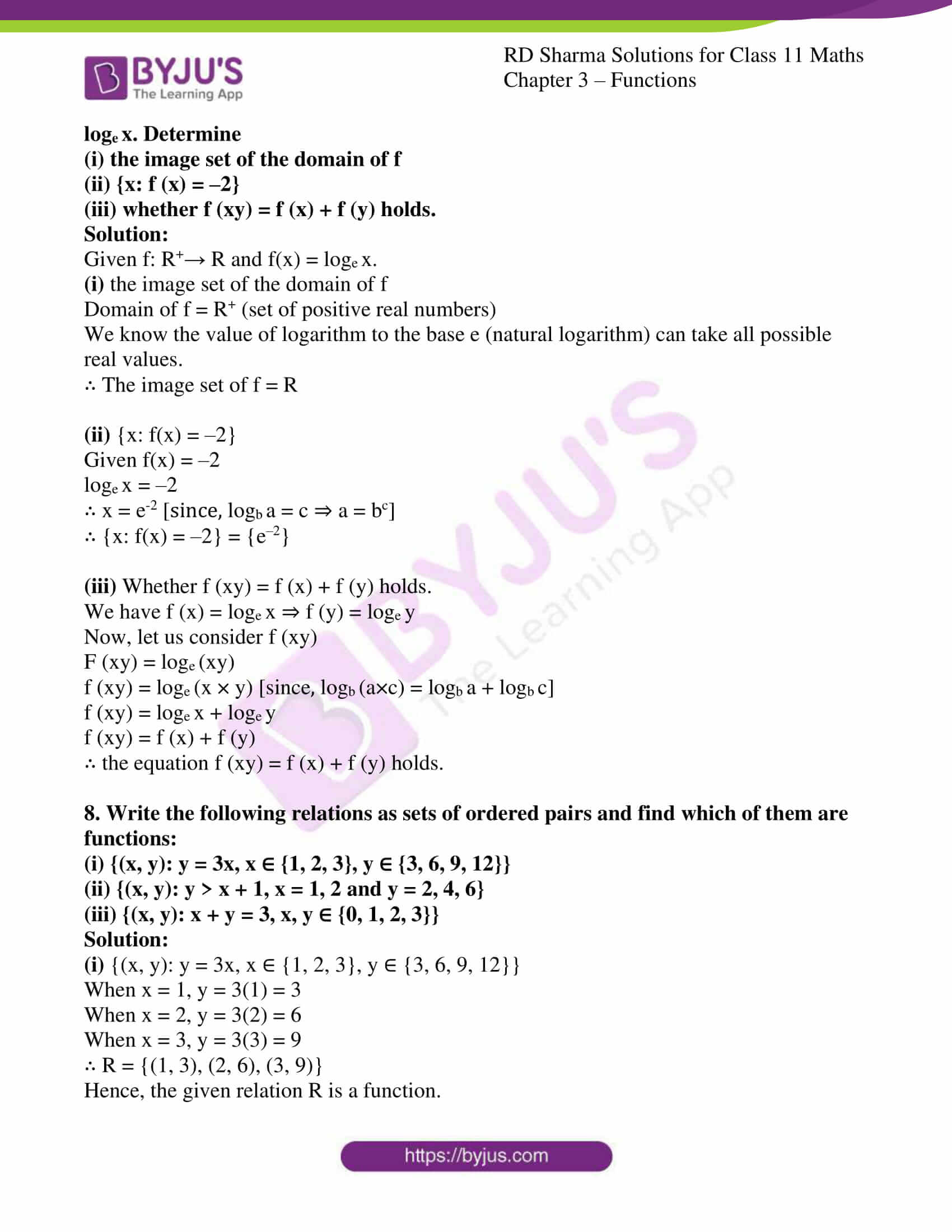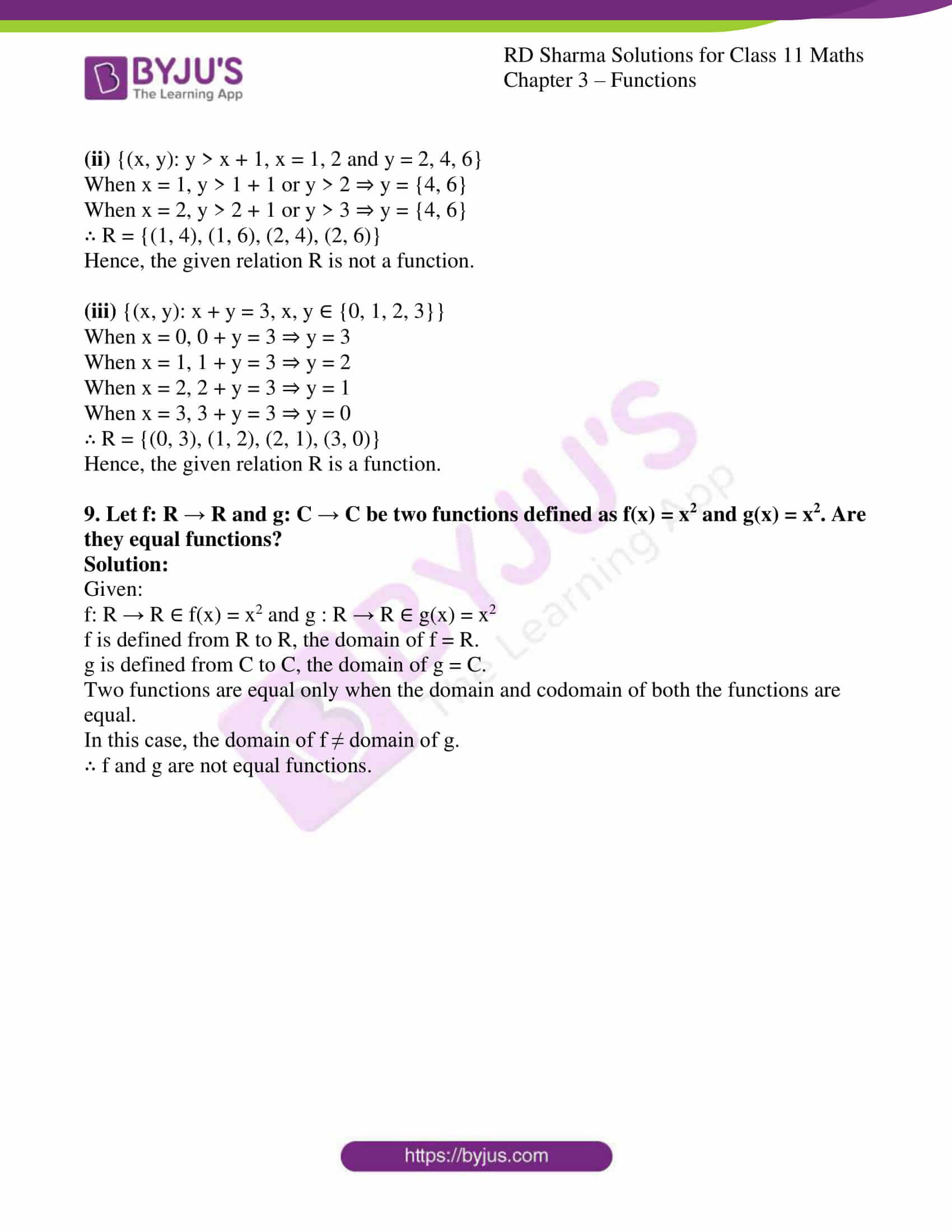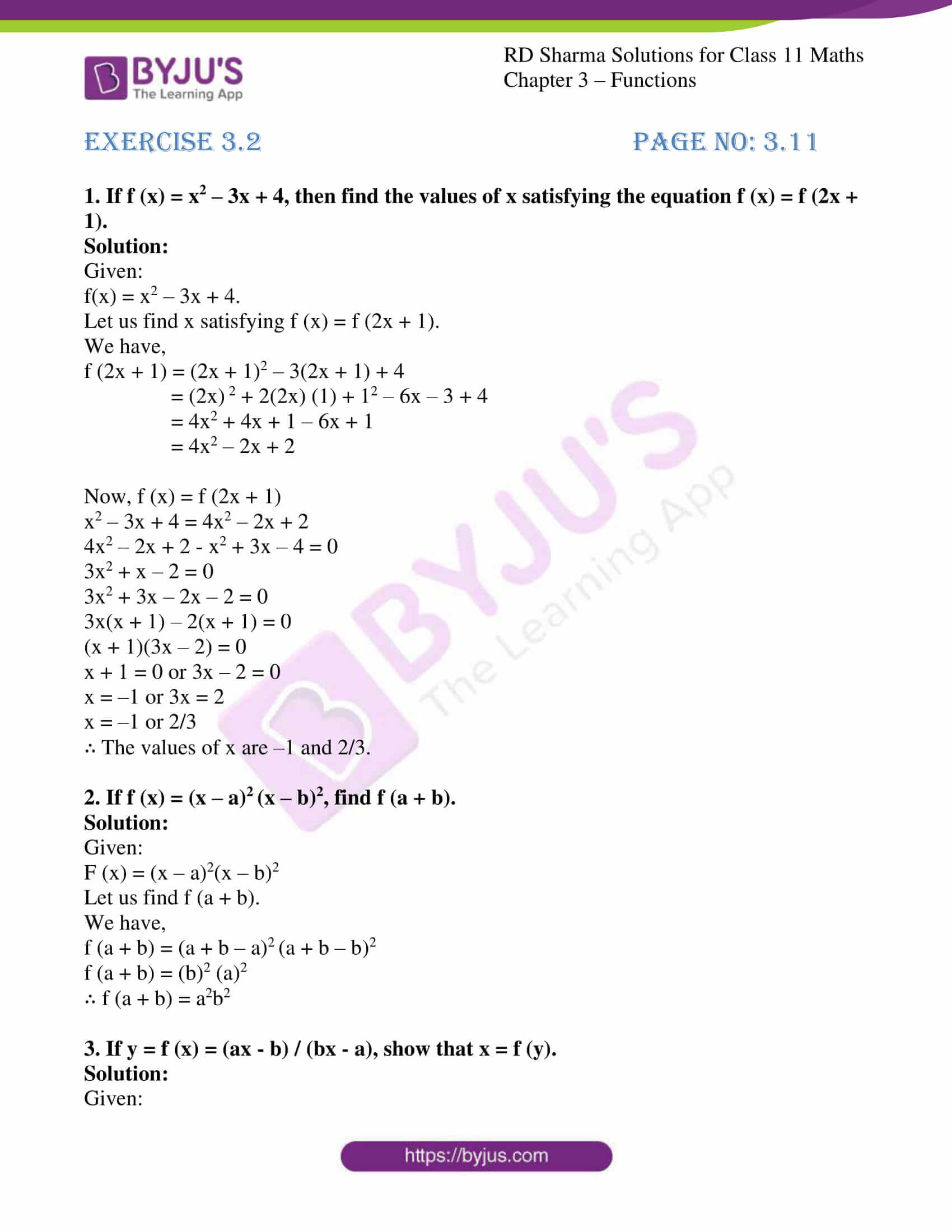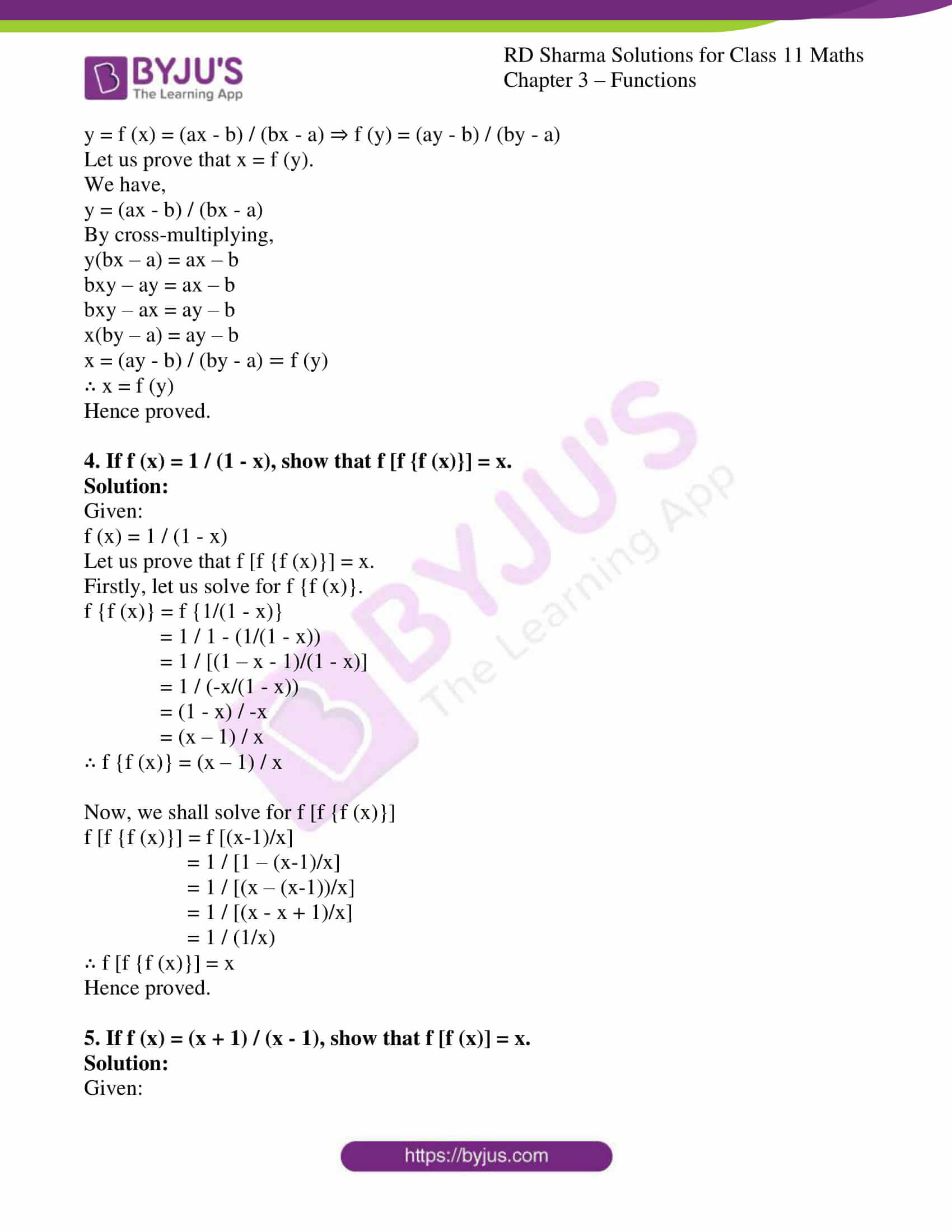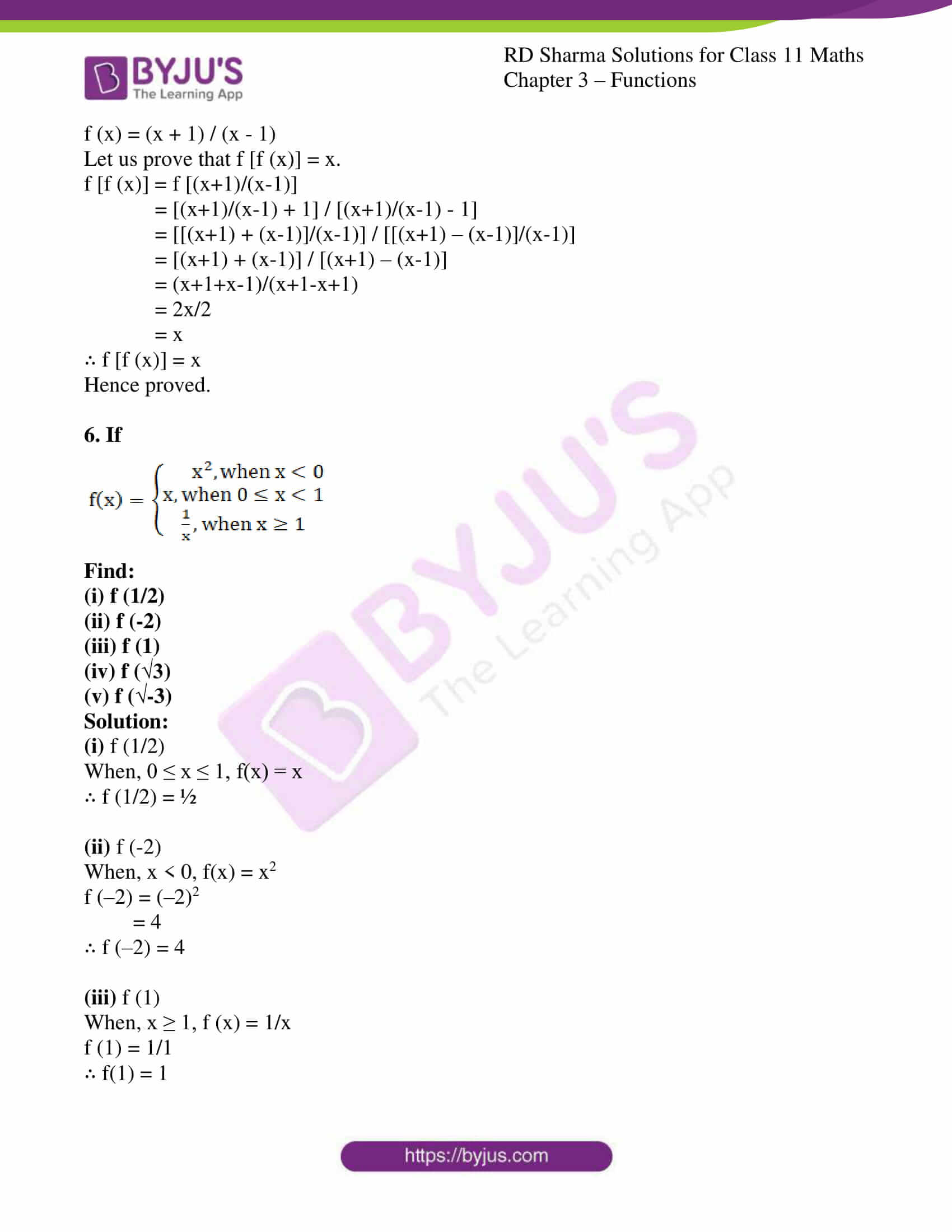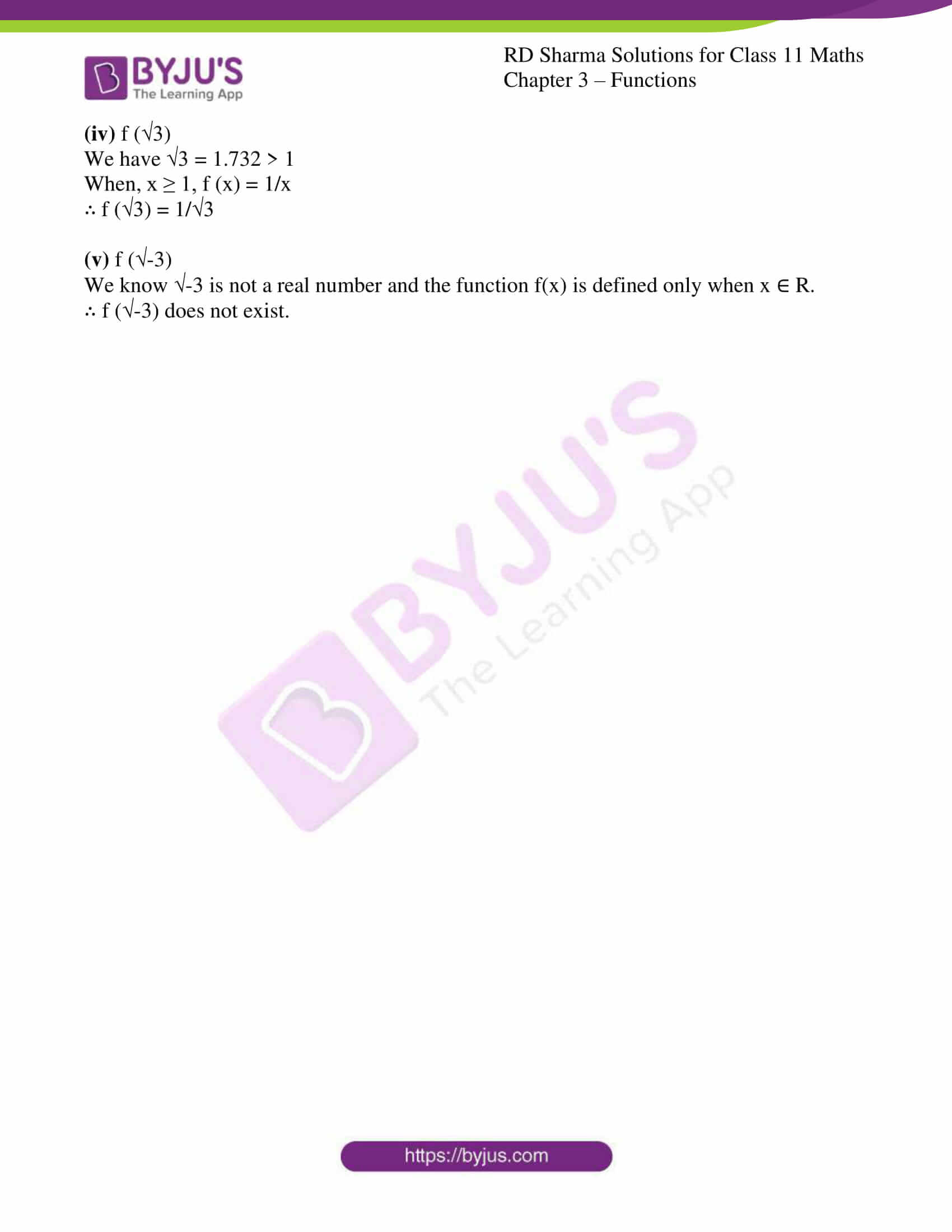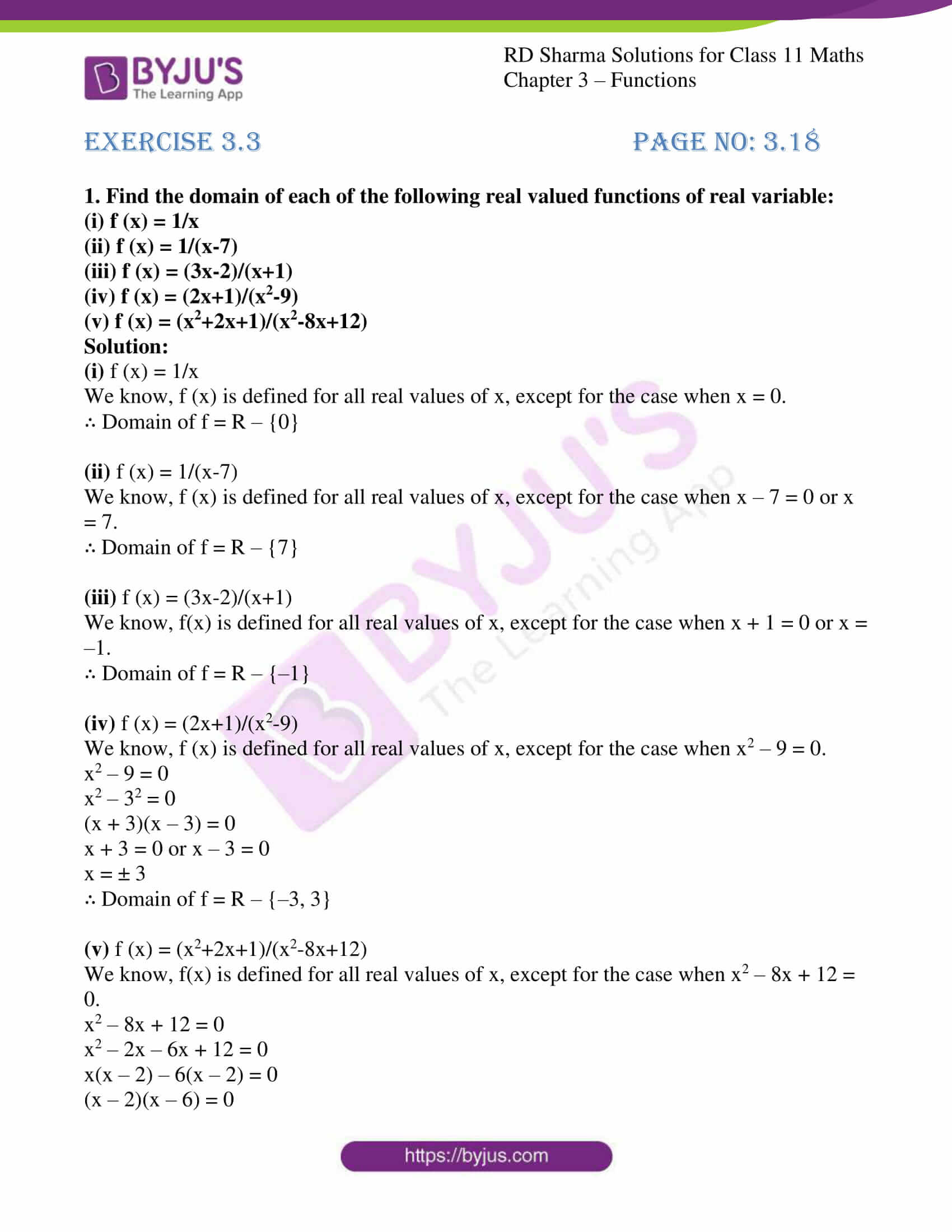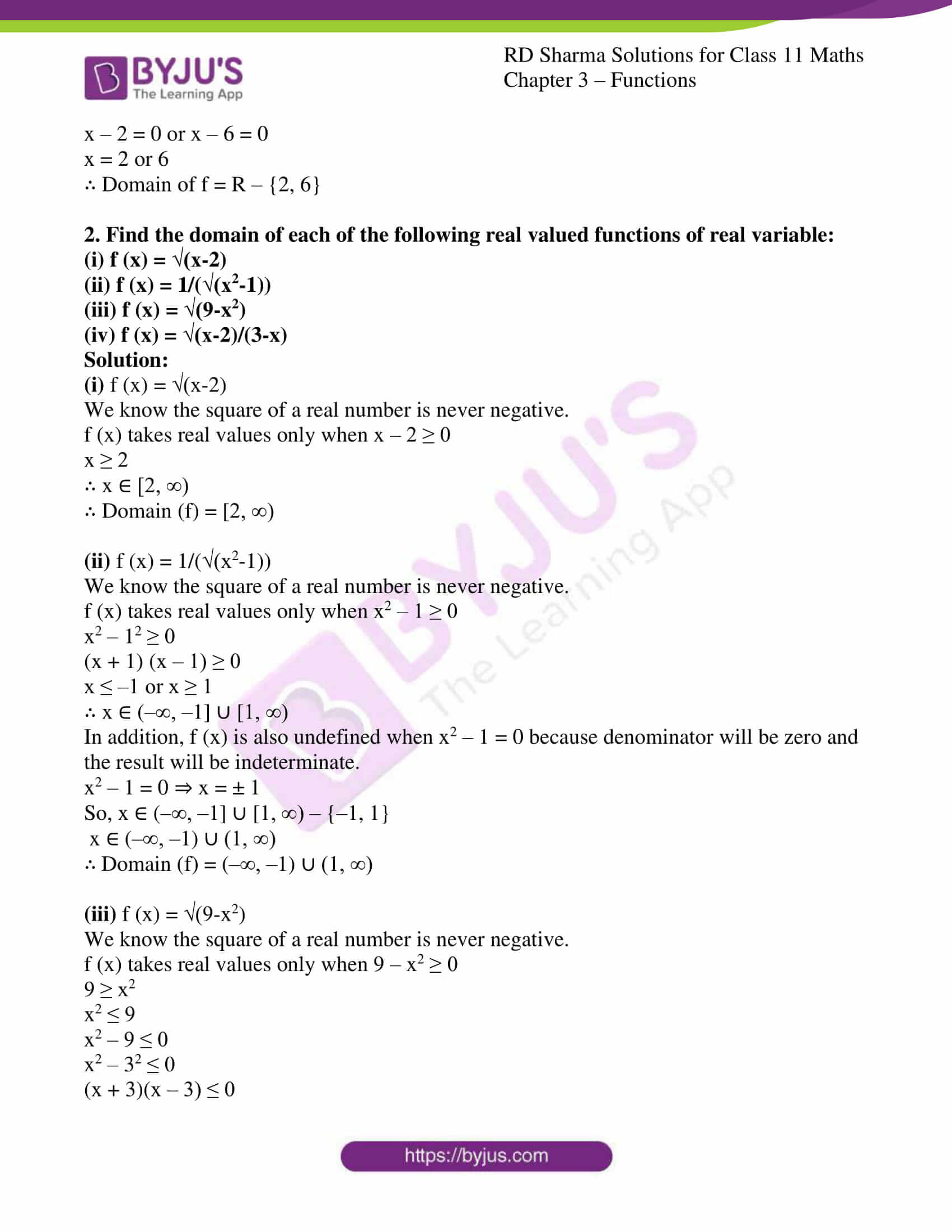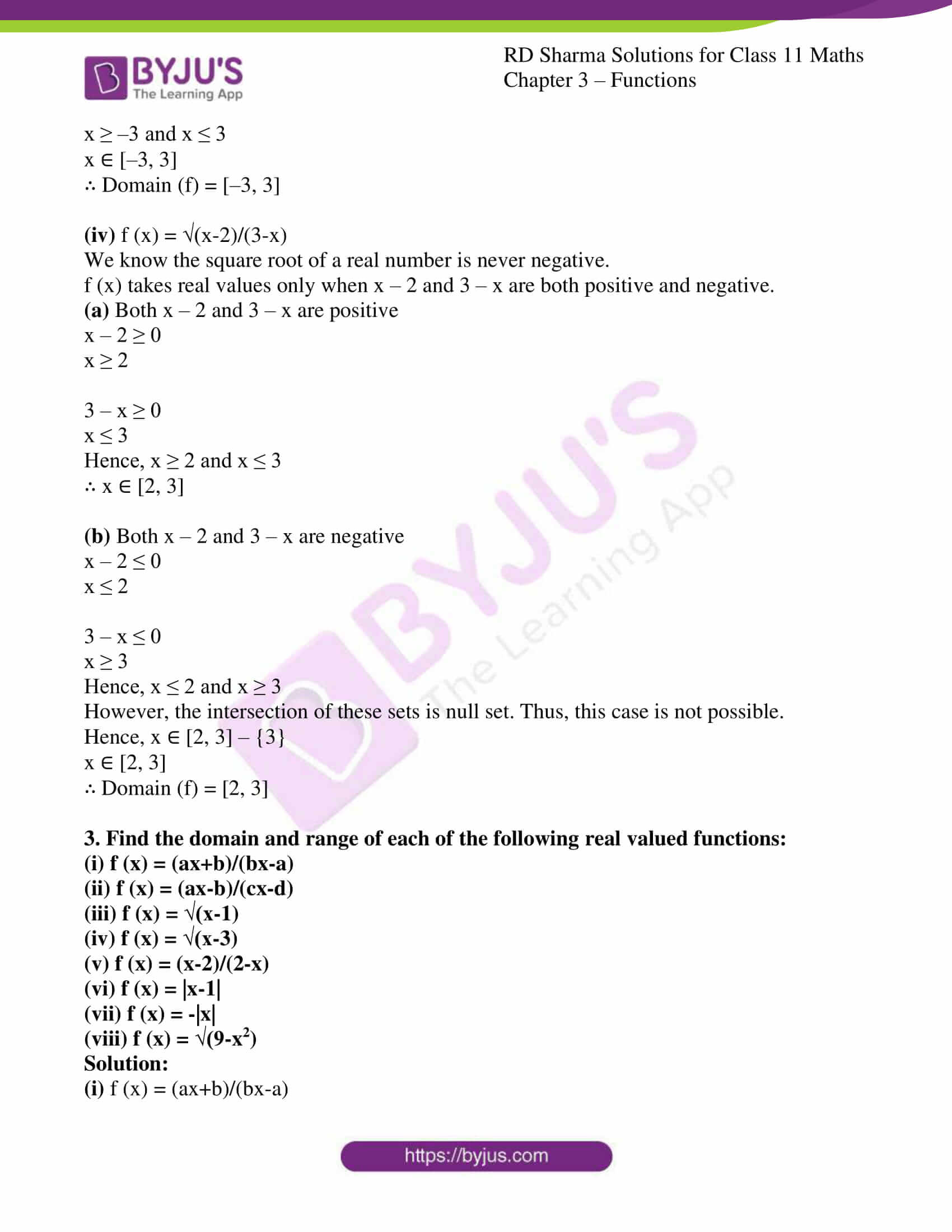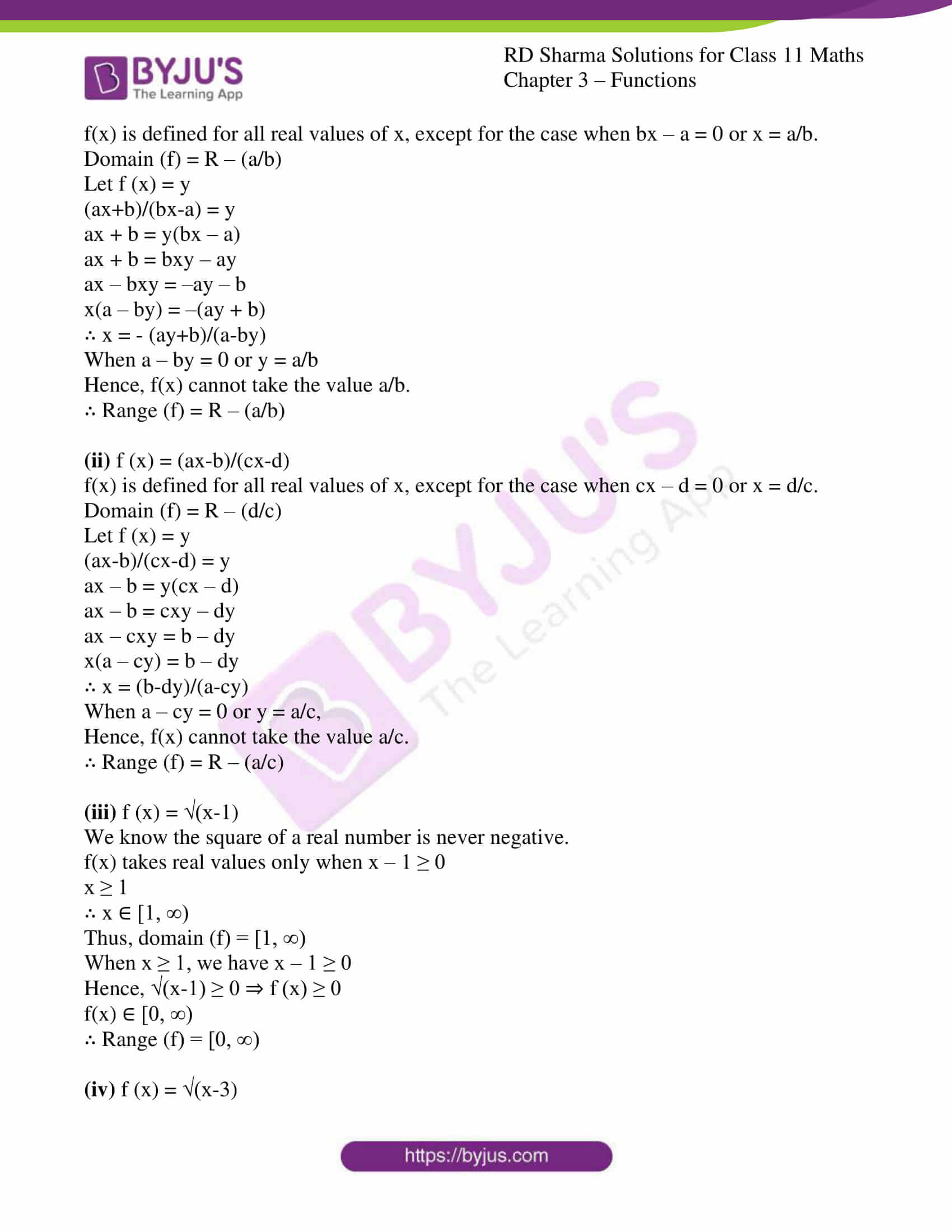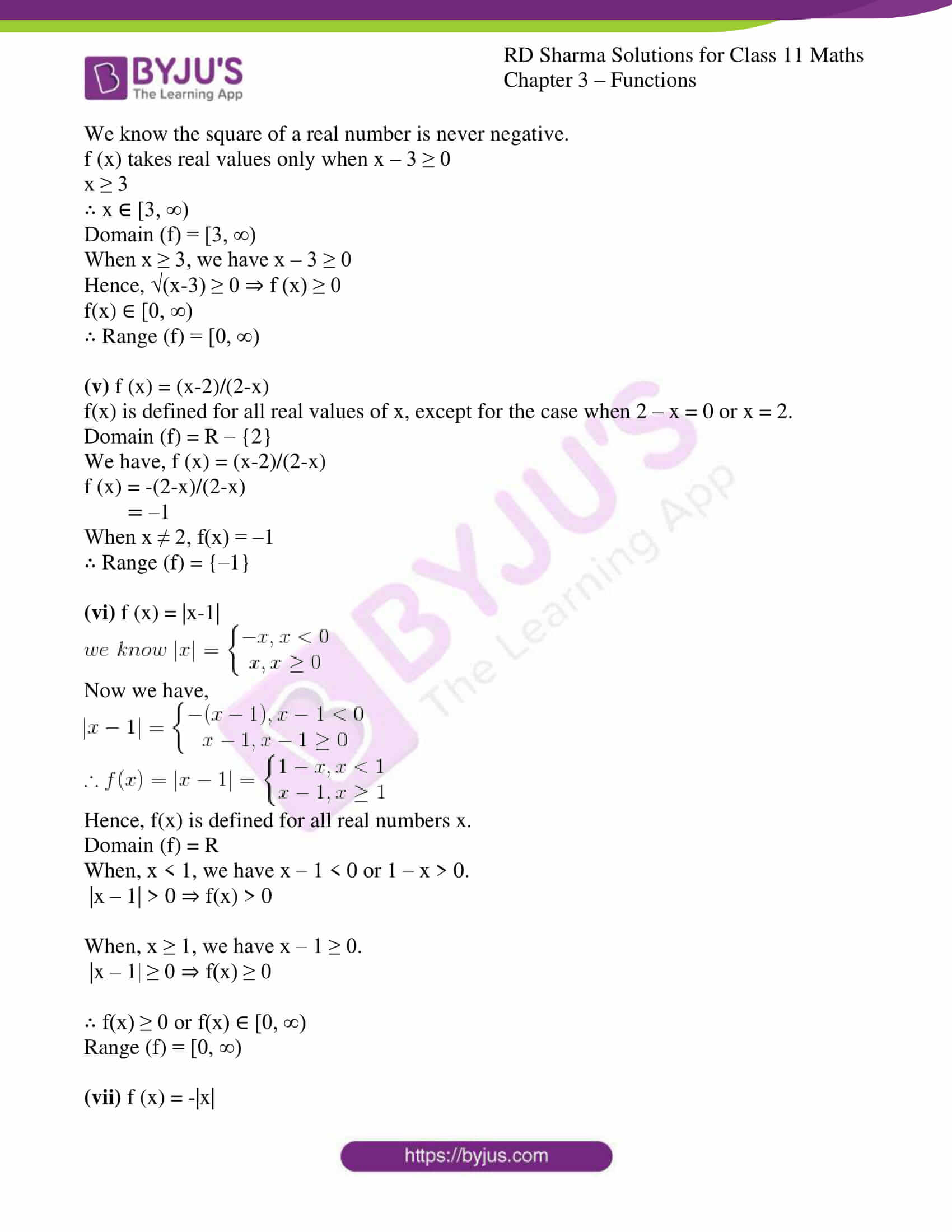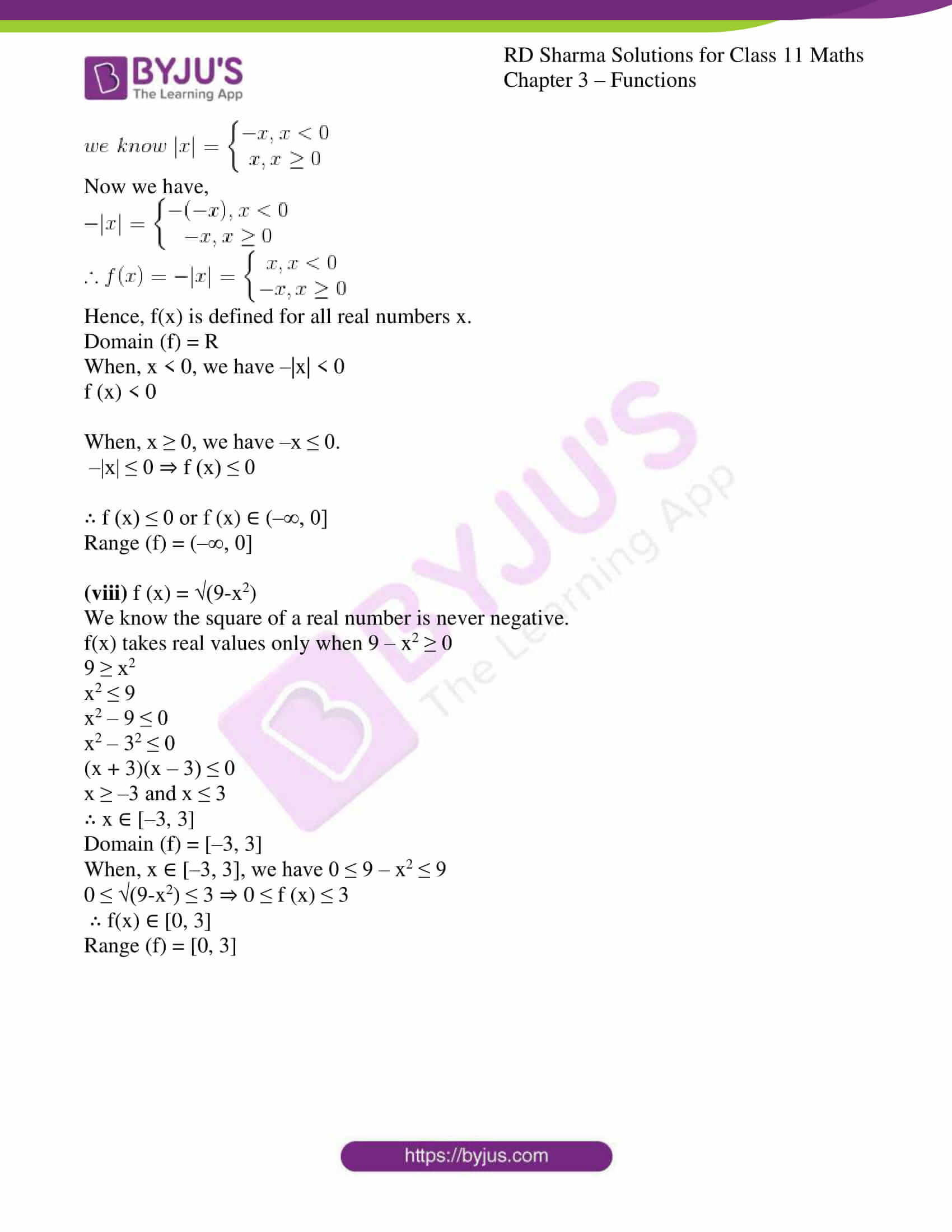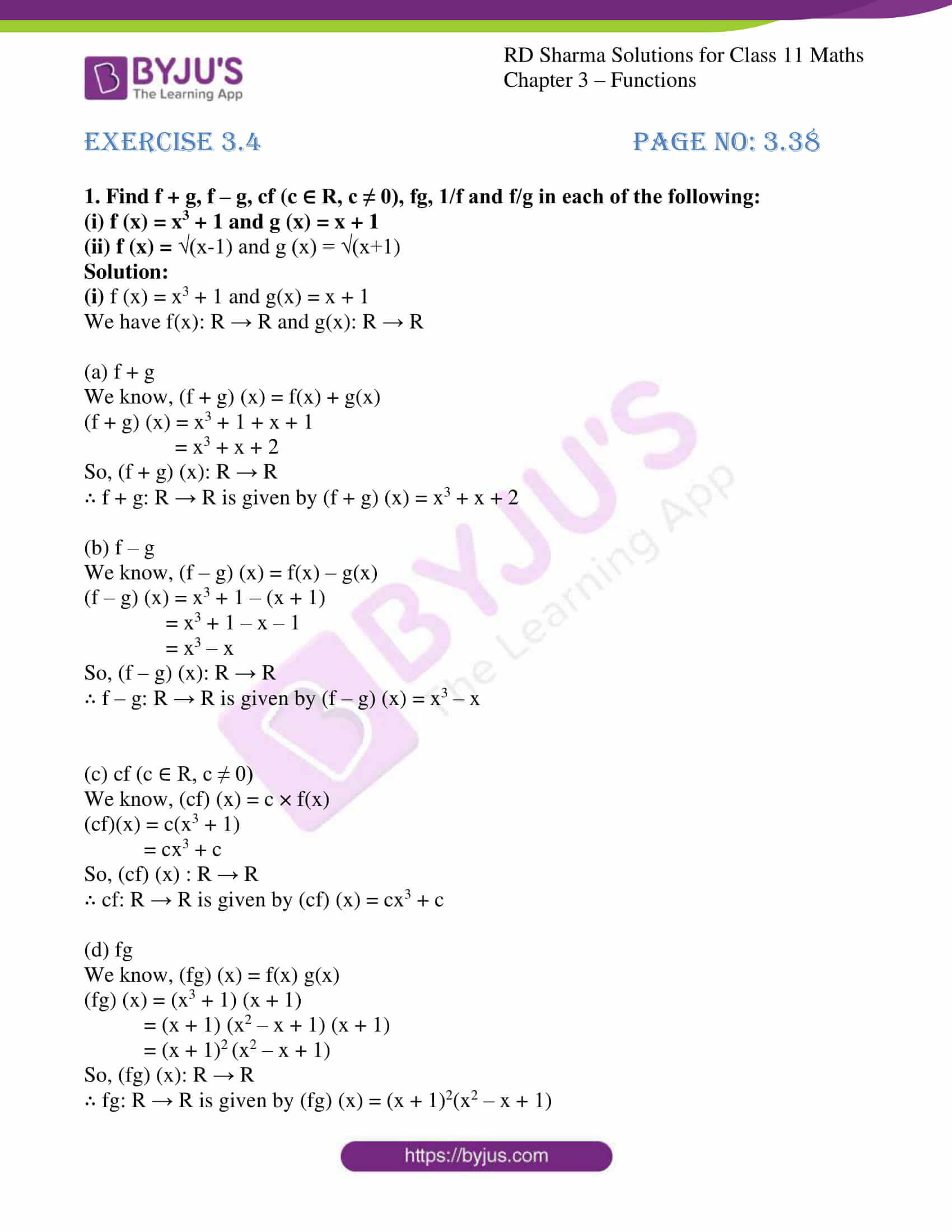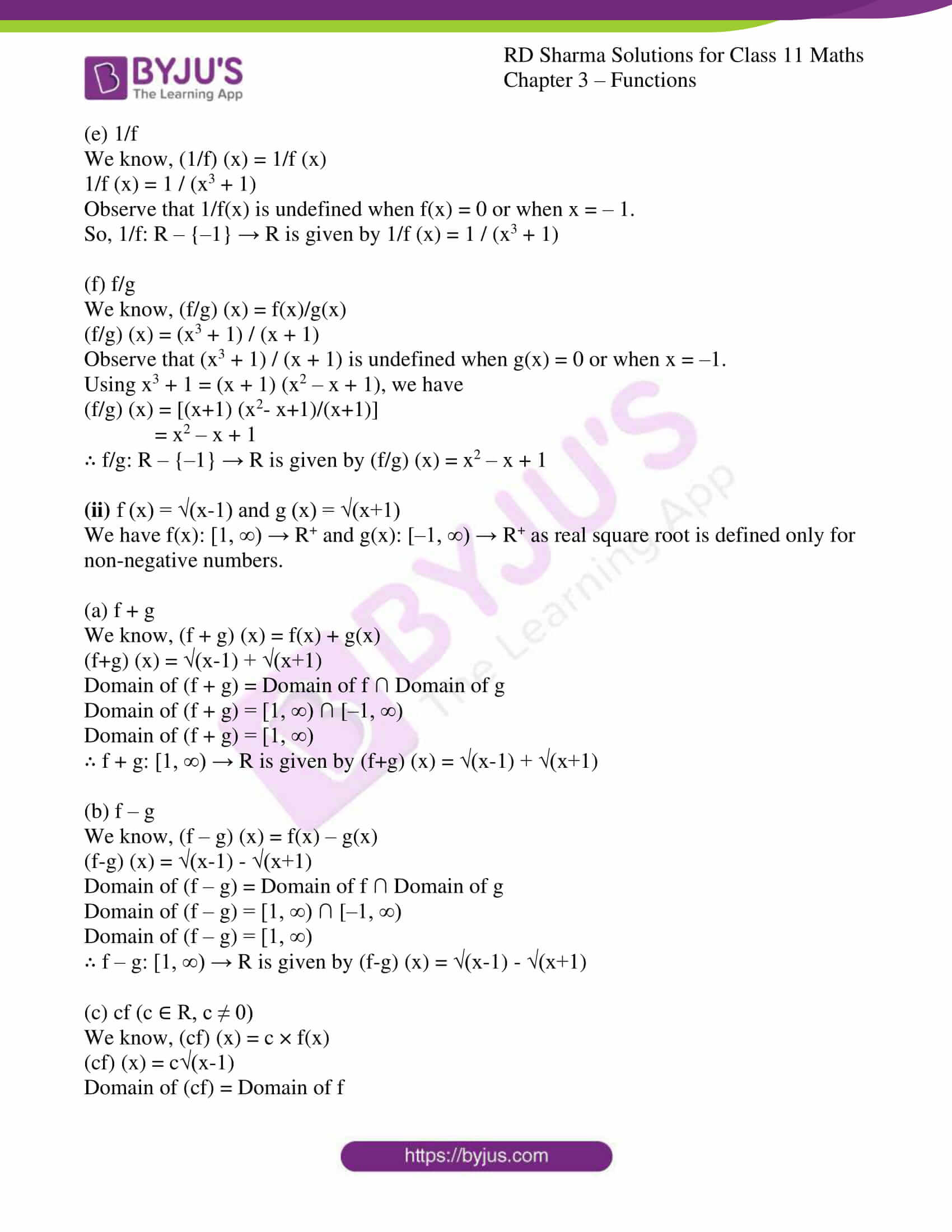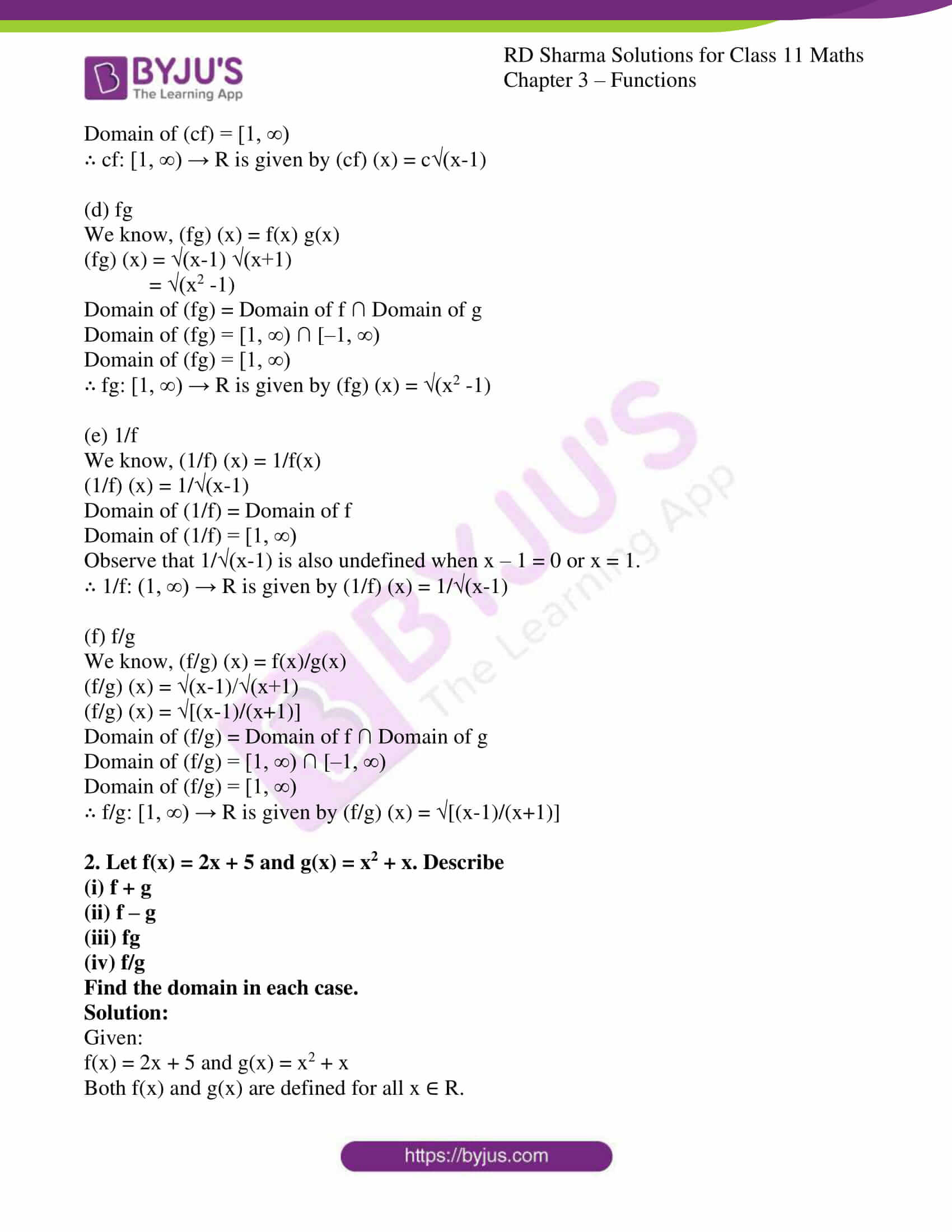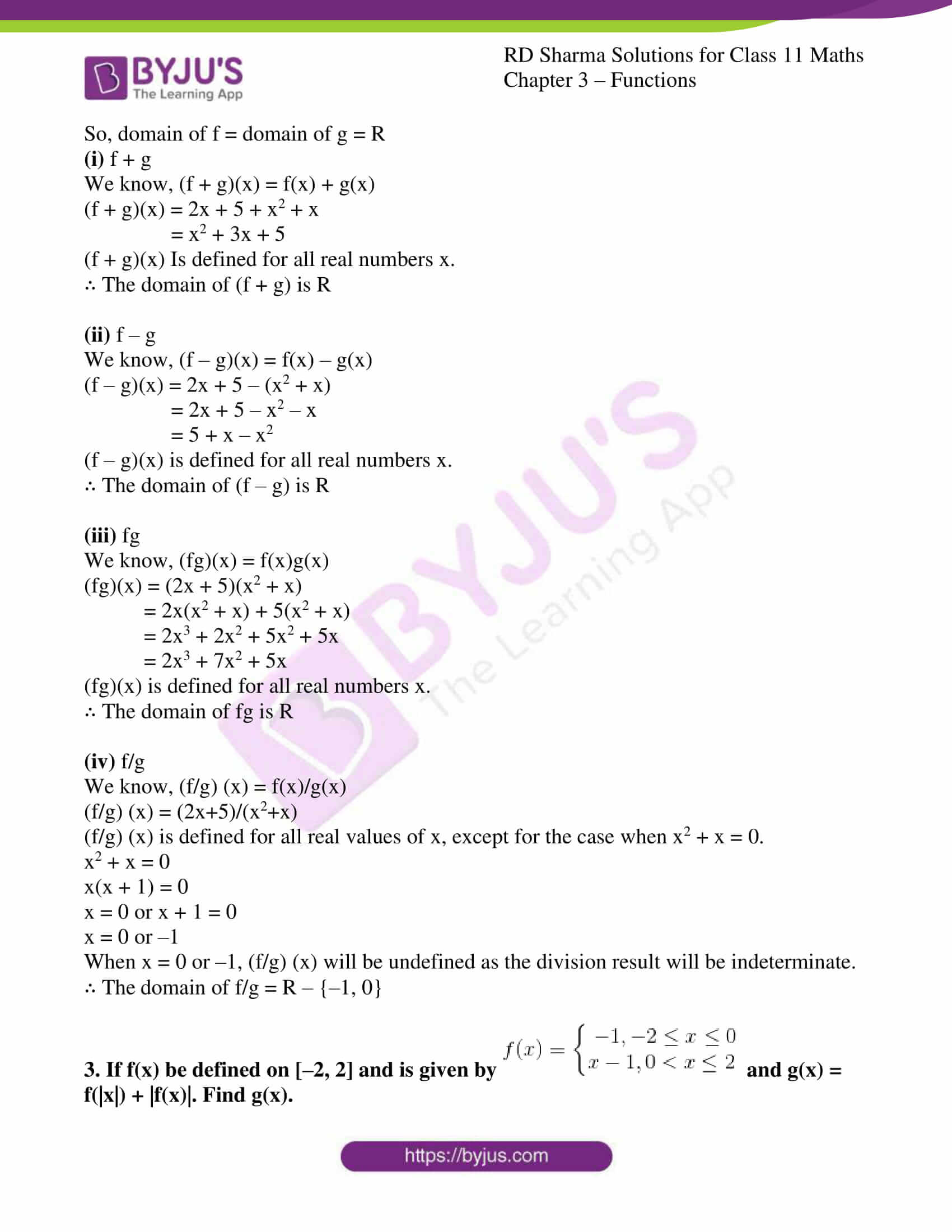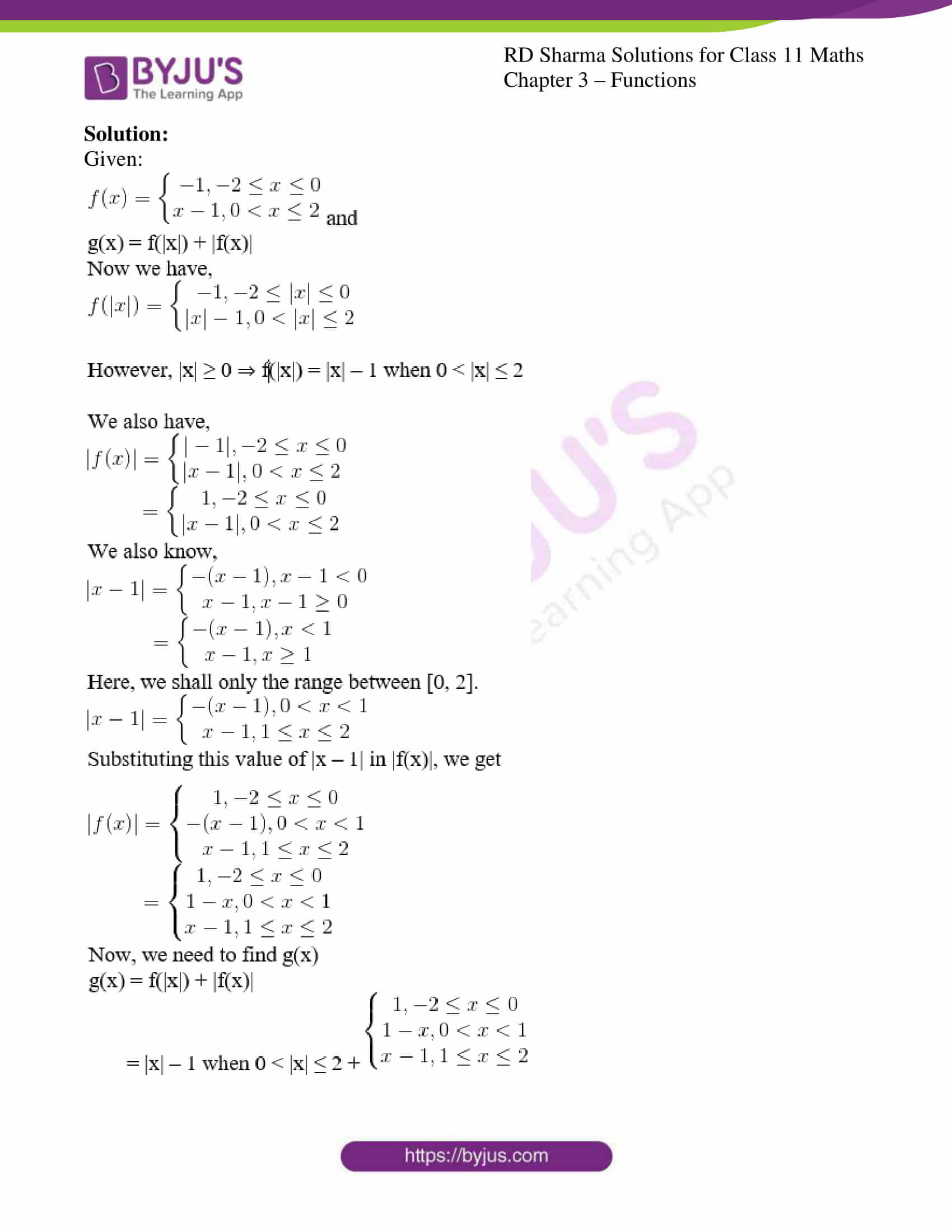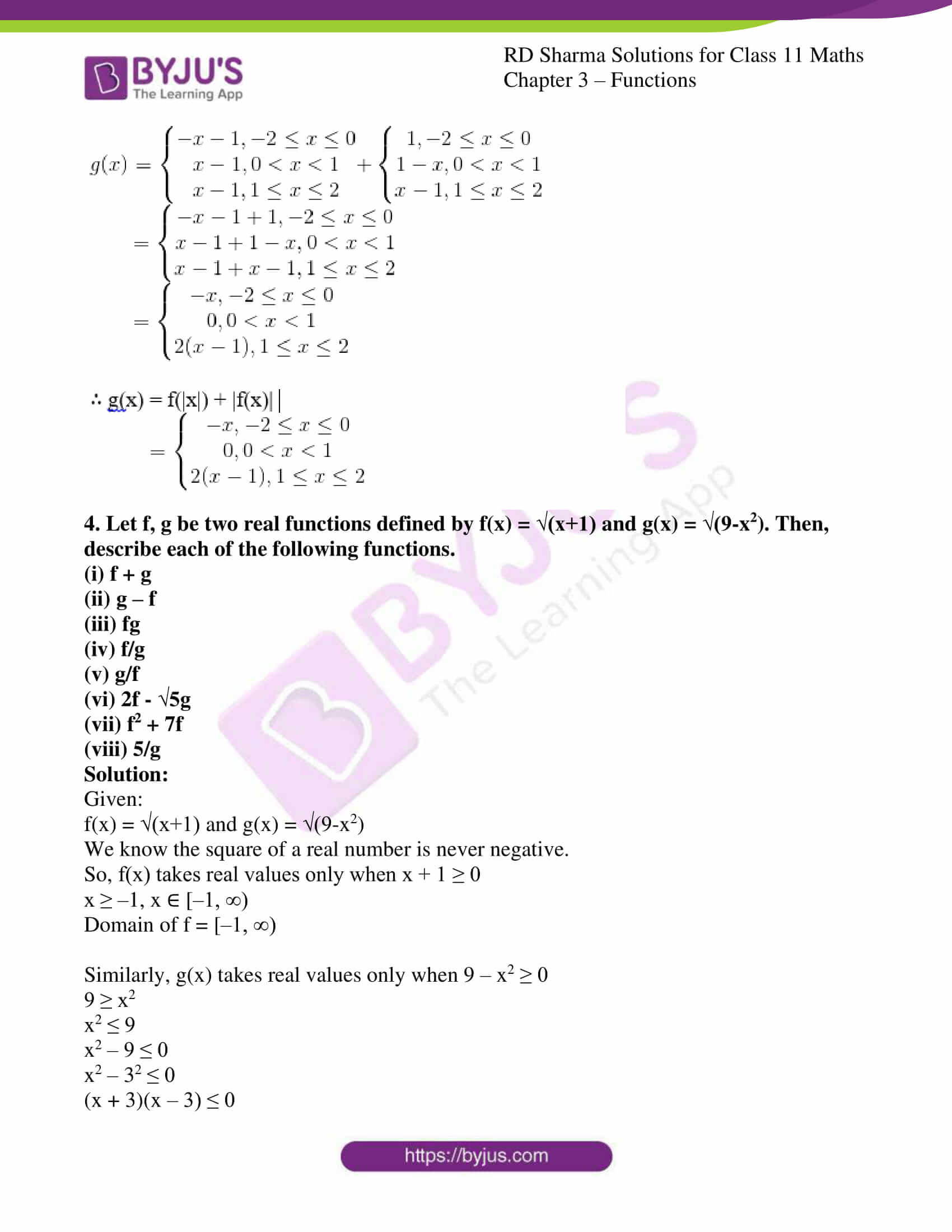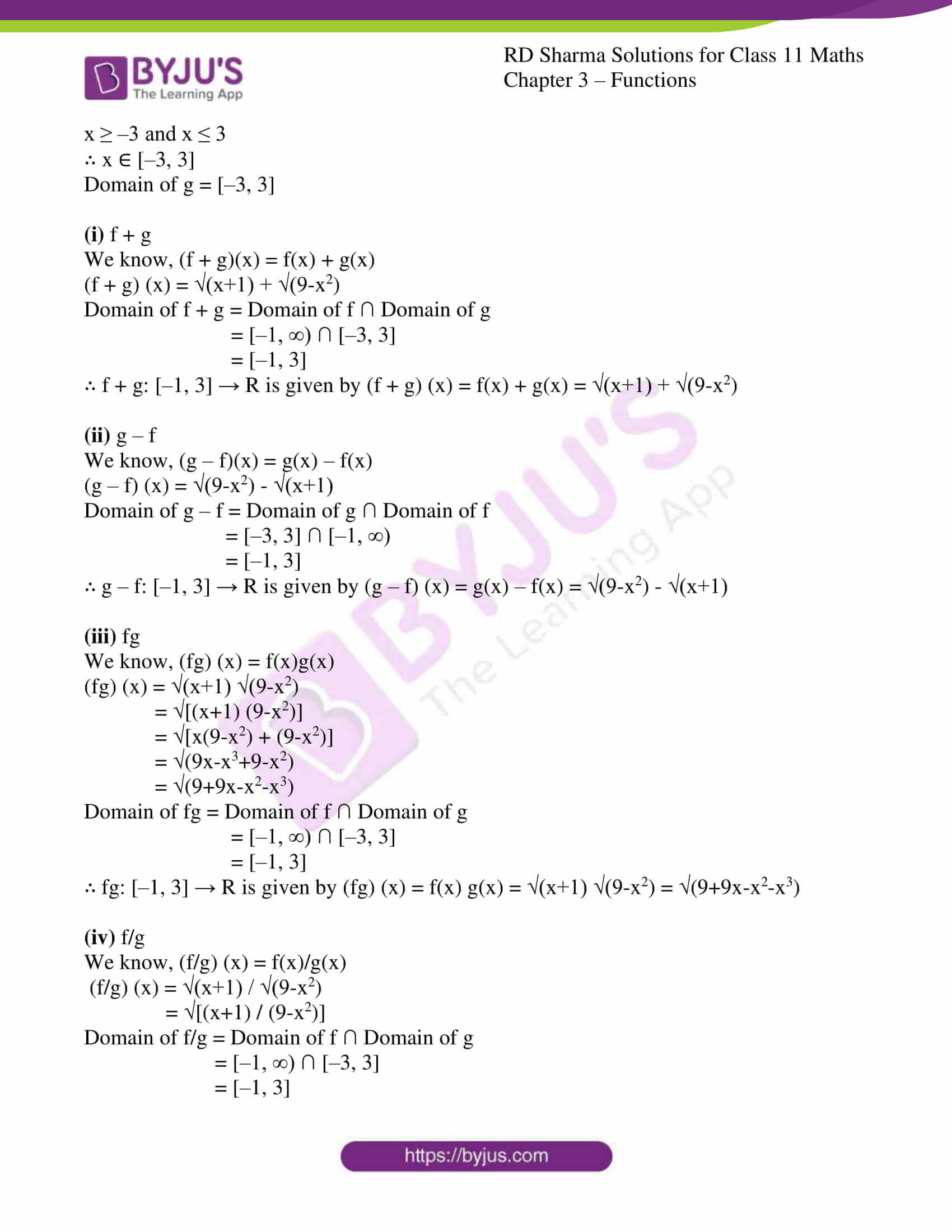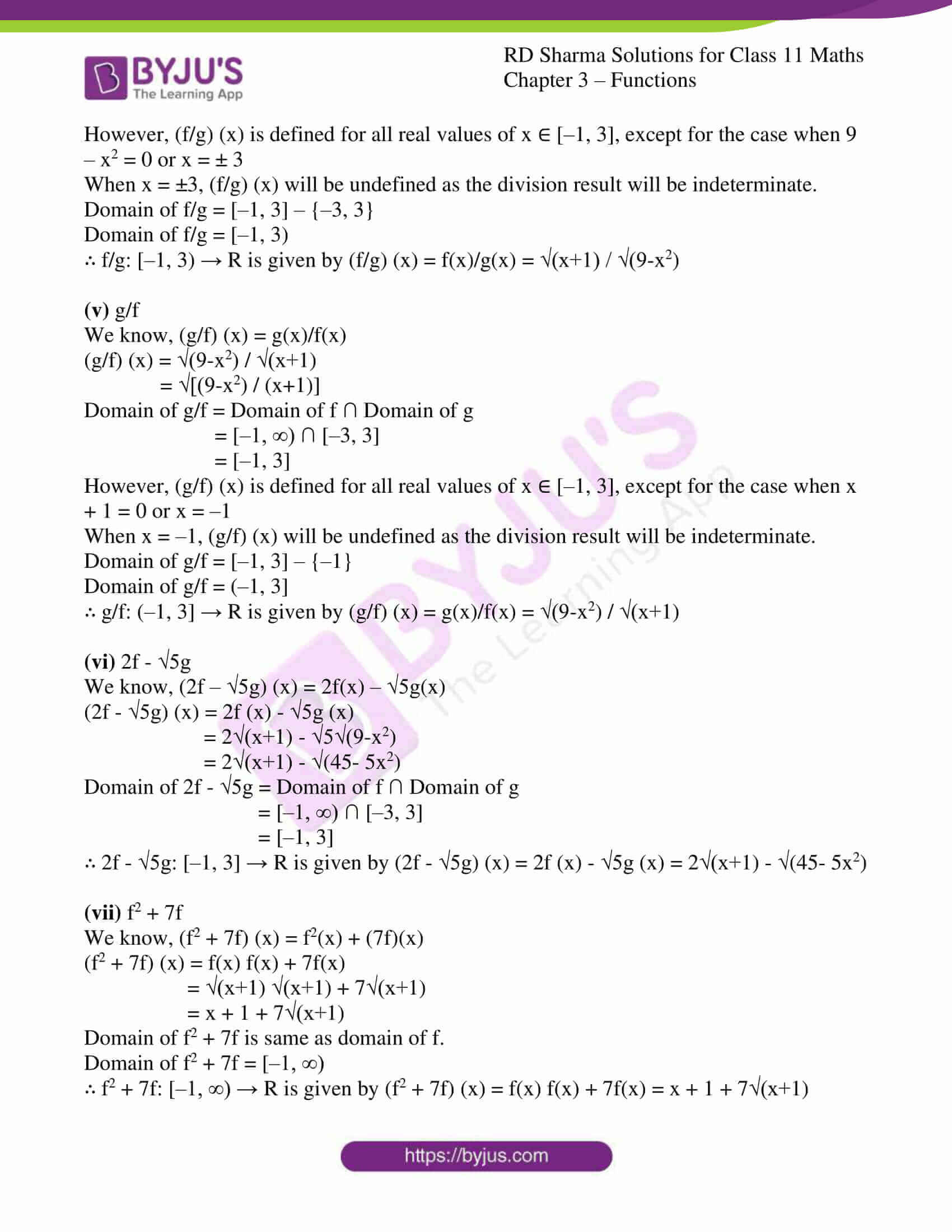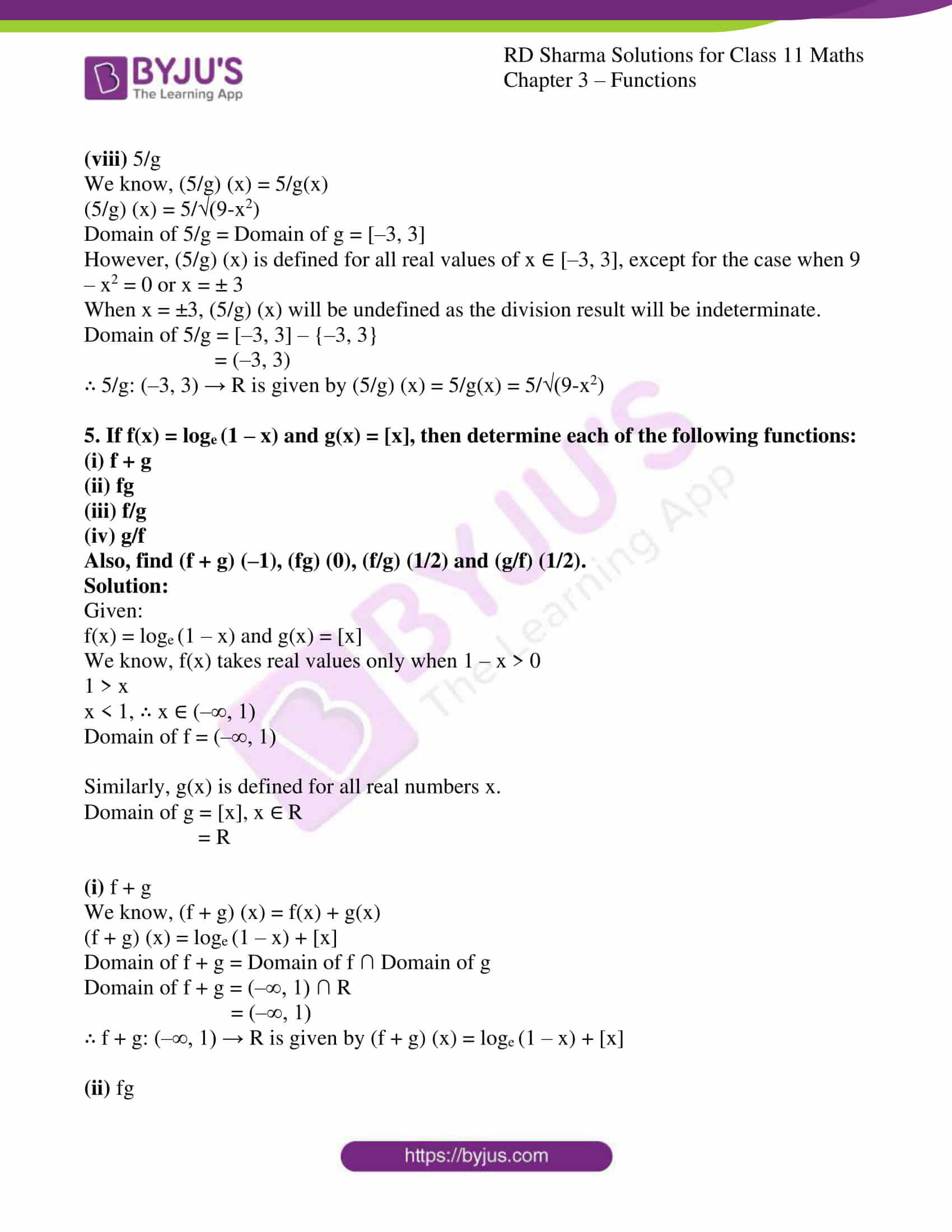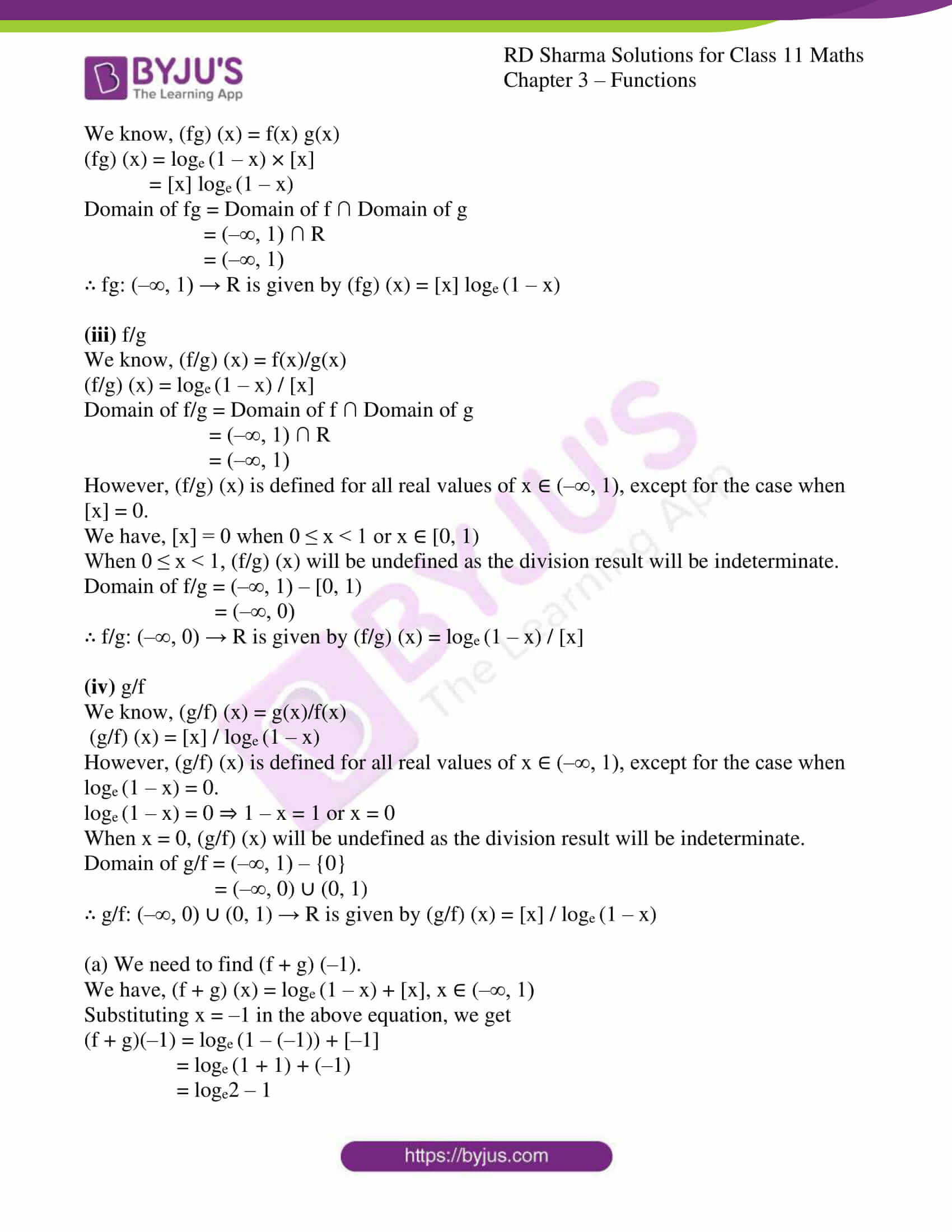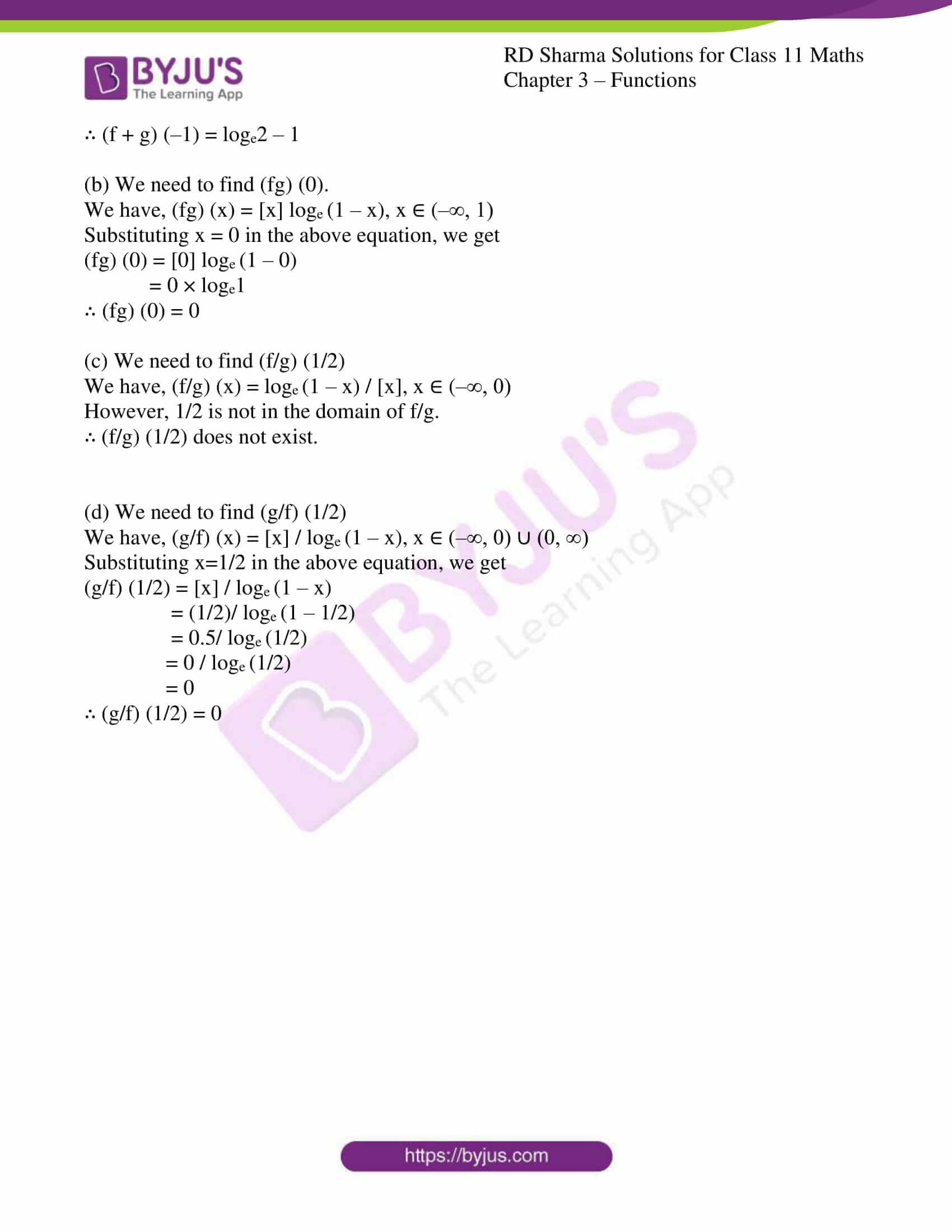### Access answers to RD Sharma Solutions for Class 11 Maths Chapter 3 – Functions

EXERCISE 3.1 PAGE NO: 3.7

1. Define a function as a set of ordered pairs.

Solution:

Let A and B be two non-empty sets. A relation from A to B, i.e., a subset of A×B, is called a function (or a mapping) from A to B, if

(i) for each a ∈ A there exists b ∈ B such that (a, b) ∈ f

(ii) (a, b) ∈ f and (a, c) ∈ f ⇒ b = c

2. Define a function as a correspondence between two sets.

Solution:

Let A and B be two non-empty sets. Then a function ‘f’ from set A to B is a rule or method or correspondence which associates elements of set A to elements of set B such that:

(i) all elements of set A are associated to elements in set B.

(ii) an element of set A is associated to a unique element in set B.

3. What is the fundamental difference between a relation and a function? Is every relation a function?

Solution:

Let ‘f’ be a function and R be a relation defined from set X to set Y.

The domain of the relation R might be a subset of the set X, but the domain of the function f must be equal to X. This is because each element of the domain of a function must have an element associated with it, whereas this is not necessary for a relation.

In relation, one element of X might be associated with one or more elements of Y, while it must be associated with only one element of Y in a function.

Thus, not every relation is a function. However, every function is necessarily a relation.

4. Let A = {–2, –1, 0, 1, 2} and f: A → Z be a function defined by f(x) = x2 – 2x – 3. Find:
(i) range of f i.e. f (A)
(ii) pre-images of 6, –3 and 5

Solution:

Given:

A = {–2, –1, 0, 1, 2}

f : A → Z such that f(x) = x2 – 2x – 3

(i) Range of f i.e. f (A)

A is the domain of the function f. Hence, range is the set of elements f(x) for all x ∈ A.

Substituting x = –2 in f(x), we get

f(–2) = (–2)2 – 2(–2) – 3

= 4 + 4 – 3

= 5

Substituting x = –1 in f(x), we get

f(–1) = (–1)2 – 2(–1) – 3

= 1 + 2 – 3

= 0

Substituting x = 0 in f(x), we get

f(0) = (0)2 – 2(0) – 3

= 0 – 0 – 3

= – 3

Substituting x = 1 in f(x), we get

f(1) = 12 – 2(1) – 3

= 1 – 2 – 3

= – 4

Substituting x = 2 in f(x), we get

f(2) = 22 – 2(2) – 3

= 4 – 4 – 3

= –3

Thus, the range of f is {-4, -3, 0, 5}.

(ii) pre-images of 6, –3 and 5

Let x be the pre-image of 6 ⇒ f(x) = 6

x2 – 2x – 3 = 6

x2 – 2x – 9 = 0

x = [-(-2) ± ((-2)2 – 4(1) (-9))] / 2(1)

= [2 ± (4+36)] / 2

= [2 ± 40] / 2

= 1 ± 10

However, 1 ± 10 ∉ A

Thus, there exists no pre-image of 6.

Now, let x be the pre-image of –3 ⇒ f(x) = –3

x2 – 2x – 3 = –3

x2 – 2x = 0

x(x – 2) = 0

x = 0 or 2

Clearly, both 0 and 2 are elements of A.

Thus, 0 and 2 are the pre-images of –3.

Now, let x be the pre-image of 5 ⇒ f(x) = 5

x2 – 2x – 3 = 5

x2 – 2x – 8= 0

x2 – 4x + 2x – 8= 0

x(x – 4) + 2(x – 4) = 0

(x + 2)(x – 4) = 0

x = –2 or 4

However, 4 ∉ A but –2 ∈ A

Thus, –2 is the pre-images of 5.

∴ Ø, {0, 2}, -2 are the pre-images of 6, -3, 5

5. If a function f: R → R be defined by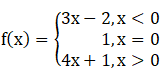Find: f (1), f (–1), f (0), f (2).

Solution:

Given:

Let us find f (1), f (–1), f (0) and f (2).

When x > 0, f (x) = 4x + 1

Substituting x = 1 in the above equation, we get

f (1) = 4(1) + 1

= 4 + 1

= 5

When x < 0, f(x) = 3x – 2

Substituting x = –1 in the above equation, we get

f (–1) = 3(–1) – 2

= –3 – 2

= –5

When x = 0, f(x) = 1

Substituting x = 0 in the above equation, we get

f (0) = 1

When x > 0, f(x) = 4x + 1

Substituting x = 2 in the above equation, we get

f (2) = 4(2) + 1

= 8 + 1

= 9

∴ f (1) = 5, f (–1) = –5, f (0) = 1 and f (2) = 9.

6. A function f: R → R is defined by f(x) = x2. Determine
(i) range of f
(ii) {x: f(x) = 4}
(iii) {y: f(y) = –1}

Solution:

Given:

f : R → R and f(x) = x2.

(i) range of f

Domain of f = R (set of real numbers)

We know that the square of a real number is always positive or equal to zero.

∴ range of f = R+∪ {0}

(ii) {x: f(x) = 4}

Given:

f(x) = 4

we know, x2 = 4

x2 – 4 = 0

(x – 2)(x + 2) = 0

∴ x = ± 2

∴ {x: f(x) = 4} = {–2, 2}

(iii) {y: f(y) = –1}

Given:

f(y) = –1

y2 = –1

However, the domain of f is R, and for every real number y, the value of y2 is non-negative.

Hence, there exists no real y for which y2 = –1.

∴{y: f(y) = –1} = ∅

7. Let f: R+→ R, where R+ is the set of all positive real numbers, be such that f(x) = loge x. Determine
(i) the image set of the domain of f
(ii) {x: f (x) = –2}
(iii) whether f (xy) = f (x) + f (y) holds.

Solution:

Given f: R+→ R and f(x) = loge x.

(i) the image set of the domain of f

Domain of f = R+ (set of positive real numbers)

We know the value of logarithm to the base e (natural logarithm) can take all possible real values.

∴ The image set of f = R

(ii) {x: f(x) = –2}

Given f(x) = –2

loge x = –2

∴ x = e-2 [since, logb a = c ⇒ a = bc]

∴ {x: f(x) = –2} = {e–2}

(iii) Whether f (xy) = f (x) + f (y) holds.

We have f (x) = loge x ⇒ f (y) = loge y

Now, let us consider f (xy)

F (xy) = loge (xy)

f (xy) = loge (x × y) [since, logb (a×c) = logb a + logb c]

f (xy) = loge x + loge y

f (xy) = f (x) + f (y)

∴ the equation f (xy) = f (x) + f (y) holds.

8. Write the following relations as sets of ordered pairs and find which of them are functions:
(i) {(x, y): y = 3x, x ∈ {1, 2, 3}, y ∈ {3, 6, 9, 12}}
(ii) {(x, y): y > x + 1, x = 1, 2 and y = 2, 4, 6}
(iii) {(x, y): x + y = 3, x, y ∈ {0, 1, 2, 3}}

Solution:

(i) {(x, y): y = 3x, x ∈ {1, 2, 3}, y ∈ {3, 6, 9, 12}}

When x = 1, y = 3(1) = 3

When x = 2, y = 3(2) = 6

When x = 3, y = 3(3) = 9

∴ R = {(1, 3), (2, 6), (3, 9)}

Hence, the given relation R is a function.

(ii) {(x, y): y > x + 1, x = 1, 2 and y = 2, 4, 6}

When x = 1, y > 1 + 1 or y > 2 ⇒ y = {4, 6}

When x = 2, y > 2 + 1 or y > 3 ⇒ y = {4, 6}

∴ R = {(1, 4), (1, 6), (2, 4), (2, 6)}

Hence, the given relation R is not a function.

(iii) {(x, y): x + y = 3, x, y ∈ {0, 1, 2, 3}}

When x = 0, 0 + y = 3 ⇒ y = 3

When x = 1, 1 + y = 3 ⇒ y = 2

When x = 2, 2 + y = 3 ⇒ y = 1

When x = 3, 3 + y = 3 ⇒ y = 0

∴ R = {(0, 3), (1, 2), (2, 1), (3, 0)}

Hence, the given relation R is a function.

9. Let f: R → R and g: C → C be two functions defined as f(x) = x2 and g(x) = x2. Are they equal functions?

Solution:

Given:

f: R → R ∈ f(x) = x2 and g : R → R ∈ g(x) = x2

f is defined from R to R, the domain of f = R.

g is defined from C to C, the domain of g = C.

Two functions are equal only when the domain and codomain of both the functions are equal.

In this case, the domain of f ≠ domain of g.

∴ f and g are not equal functions.

EXERCISE 3.2 PAGE NO: 3.11

1. If f (x) = x2 – 3x + 4, then find the values of x satisfying the equation f (x) = f (2x + 1).

Solution:

Given:

f(x) = x2 – 3x + 4.

Let us find x satisfying f (x) = f (2x + 1).

We have,

f (2x + 1) = (2x + 1)2 – 3(2x + 1) + 4

= (2x) 2 + 2(2x) (1) + 12 – 6x – 3 + 4

= 4x2 + 4x + 1 – 6x + 1

= 4x2 – 2x + 2

Now, f (x) = f (2x + 1)

x2 – 3x + 4 = 4x2 – 2x + 2

4x2 – 2x + 2 – x2 + 3x – 4 = 0

3x2 + x – 2 = 0

3x2 + 3x – 2x – 2 = 0

3x(x + 1) – 2(x + 1) = 0

(x + 1)(3x – 2) = 0

x + 1 = 0 or 3x – 2 = 0

x = –1 or 3x = 2

x = –1 or 2/3

∴ The values of x are –1 and 2/3.

2. If f (x) = (x – a)2 (x – b)2, find f (a + b).

Solution:

Given:

F (x) = (x – a)2(x – b)2

Let us find f (a + b).

We have,

f (a + b) = (a + b – a)2 (a + b – b)2

f (a + b) = (b)2 (a)2

∴ f (a + b) = a2b2

3. If y = f (x) = (ax – b) / (bx – a), show that x = f (y).

Solution:

Given:

y = f (x) = (ax – b) / (bx – a) ⇒ f (y) = (ay – b) / (by – a)

Let us prove that x = f (y).

We have,

y = (ax – b) / (bx – a)

By cross-multiplying,

y(bx – a) = ax – b

bxy – ay = ax – b

bxy – ax = ay – b

x(by – a) = ay – b

x = (ay – b) / (by – a) = f (y)

∴ x = f (y)

Hence proved.

4. If f (x) = 1 / (1 – x), show that f [f {f (x)}] = x.

Solution:

Given:

f (x) = 1 / (1 – x)

Let us prove that f [f {f (x)}] = x.

Firstly, let us solve for f {f (x)}.

f {f (x)} = f {1/(1 – x)}

= 1 / 1 – (1/(1 – x))

= 1 / [(1 – x – 1)/(1 – x)]

= 1 / (-x/(1 – x))

= (1 – x) / -x

= (x – 1) / x

∴ f {f (x)} = (x – 1) / x

Now, we shall solve for f [f {f (x)}]

f [f {f (x)}] = f [(x-1)/x]

= 1 / [1 – (x-1)/x]

= 1 / [(x – (x-1))/x]

= 1 / [(x – x + 1)/x]

= 1 / (1/x)

∴ f [f {f (x)}] = x

Hence proved.

5. If f (x) = (x + 1) / (x – 1), show that f [f (x)] = x.

Solution:

Given:

f (x) = (x + 1) / (x – 1)

Let us prove that f [f (x)] = x.

f [f (x)] = f [(x+1)/(x-1)]

= [(x+1)/(x-1) + 1] / [(x+1)/(x-1) – 1]

= [[(x+1) + (x-1)]/(x-1)] / [[(x+1) – (x-1)]/(x-1)]

= [(x+1) + (x-1)] / [(x+1) – (x-1)]

= (x+1+x-1)/(x+1-x+1)

= 2x/2

= x

∴ f [f (x)] = x

Hence proved.

6. If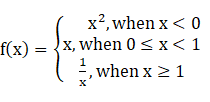Find:

(i) f (1/2)

(ii) f (-2)

(iii) f (1)

(iv) f (√3)

(v) f (√-3)

Solution:

(i) f (1/2)

When, 0 ≤ x ≤ 1, f(x) = x

∴ f (1/2) = ½

(ii) f (-2)

When, x < 0, f(x) = x2

f (–2) = (–2)2

= 4

∴ f (–2) = 4

(iii) f (1)

When, x ≥ 1, f (x) = 1/x

f (1) = 1/1

∴ f(1) = 1

(iv) f (√3)

We have √3 = 1.732 > 1

When, x ≥ 1, f (x) = 1/x

∴ f (√3) = 1/√3

(v) f (√-3)

We know √-3 is not a real number and the function f(x) is defined only when x ∈ R.

∴ f (√-3) does not exist.

EXERCISE 3.3 PAGE NO: 3.18

1. Find the domain of each of the following real valued functions of real variable:

(i) f (x) = 1/x

(ii) f (x) = 1/(x-7)

(iii) f (x) = (3x-2)/(x+1)

(iv) f (x) = (2x+1)/(x2-9)

(v) f (x) = (x2+2x+1)/(x2-8x+12)

Solution:

(i) f (x) = 1/x

We know, f (x) is defined for all real values of x, except for the case when x = 0.

∴ Domain of f = R – {0}

(ii) f (x) = 1/(x-7)

We know, f (x) is defined for all real values of x, except for the case when x – 7 = 0 or x = 7.

∴ Domain of f = R – {7}

(iii) f (x) = (3x-2)/(x+1)

We know, f(x) is defined for all real values of x, except for the case when x + 1 = 0 or x = –1.

∴ Domain of f = R – {–1}

(iv) f (x) = (2x+1)/(x2-9)

We know, f (x) is defined for all real values of x, except for the case when x2 – 9 = 0.

x2 – 9 = 0

x2 – 32 = 0

(x + 3)(x – 3) = 0

x + 3 = 0 or x – 3 = 0

x = ± 3

∴ Domain of f = R – {–3, 3}

(v) f (x) = (x2+2x+1)/(x2-8x+12)

We know, f(x) is defined for all real values of x, except for the case when x2 – 8x + 12 = 0.

x2 – 8x + 12 = 0

x2 – 2x – 6x + 12 = 0

x(x – 2) – 6(x – 2) = 0

(x – 2)(x – 6) = 0

x – 2 = 0 or x – 6 = 0

x = 2 or 6

∴ Domain of f = R – {2, 6}

2. Find the domain of each of the following real valued functions of real variable:

(i) f (x) = √(x-2)

(ii) f (x) = 1/(√(x2-1))

(iii) f (x) = √(9-x2)

(iv) f (x) = √(x-2)/(3-x)

Solution:

(i) f (x) = √(x-2)

We know the square of a real number is never negative.

f (x) takes real values only when x – 2 ≥ 0

x ≥ 2

∴ x ∈ [2, ∞)

∴ Domain (f) = [2, ∞)

(ii) f (x) = 1/(√(x2-1))

We know the square of a real number is never negative.

f (x) takes real values only when x2 – 1 ≥ 0

x2 – 12 ≥ 0

(x + 1) (x – 1) ≥ 0

x ≤ –1 or x ≥ 1

∴ x ∈ (–∞, –1] ∪ [1, ∞)

In addition, f (x) is also undefined when x2 – 1 = 0 because denominator will be zero and the result will be indeterminate.

x2 – 1 = 0 ⇒ x = ± 1

So, x ∈ (–∞, –1] ∪ [1, ∞) – {–1, 1}

x ∈ (–∞, –1) ∪ (1, ∞)

∴ Domain (f) = (–∞, –1) ∪ (1, ∞)

(iii) f (x) = √(9-x2)

We know the square of a real number is never negative.

f (x) takes real values only when 9 – x2 ≥ 0

9 ≥ x2

x2 ≤ 9

x2 – 9 ≤ 0

x2 – 32 ≤ 0

(x + 3)(x – 3) ≤ 0

x ≥ –3 and x ≤ 3

x ∈ [–3, 3]

∴ Domain (f) = [–3, 3]

(iv) f (x) = √(x-2)/(3-x)

We know the square root of a real number is never negative.

f (x) takes real values only when x – 2 and 3 – x are both positive and negative.

(a) Both x – 2 and 3 – x are positive

x – 2 ≥ 0

x ≥ 2

3 – x ≥ 0

x ≤ 3

Hence, x ≥ 2 and x ≤ 3

∴ x ∈ [2, 3]

(b) Both x – 2 and 3 – x are negative

x – 2 ≤ 0

x ≤ 2

3 – x ≤ 0

x ≥ 3

Hence, x ≤ 2 and x ≥ 3

However, the intersection of these sets is null set. Thus, this case is not possible.

Hence, x ∈ [2, 3] – {3}

x ∈ [2, 3]

∴ Domain (f) = [2, 3]

3. Find the domain and range of each of the following real valued functions:

(i) f (x) = (ax+b)/(bx-a)

(ii) f (x) = (ax-b)/(cx-d)

(iii) f (x) = √(x-1)

(iv) f (x) = √(x-3)

(v) f (x) = (x-2)/(2-x)

(vi) f (x) = |x-1|

(vii) f (x) = -|x|

(viii) f (x) = √(9-x2)

Solution:

(i) f (x) = (ax+b)/(bx-a)

f(x) is defined for all real values of x, except for the case when bx – a = 0 or x = a/b.

Domain (f) = R – (a/b)

Let f (x) = y

(ax+b)/(bx-a) = y

ax + b = y(bx – a)

ax + b = bxy – ay

ax – bxy = –ay – b

x(a – by) = –(ay + b)

∴ x = – (ay+b)/(a-by)

When a – by = 0 or y = a/b

Hence, f(x) cannot take the value a/b.

∴ Range (f) = R – (a/b)

(ii) f (x) = (ax-b)/(cx-d)

f(x) is defined for all real values of x, except for the case when cx – d = 0 or x = d/c. Domain (f) = R – (d/c)

Let f (x) = y

(ax-b)/(cx-d) = y

ax – b = y(cx – d)

ax – b = cxy – dy

ax – cxy = b – dy

x(a – cy) = b – dy

∴ x = (b-dy)/(a-cy)

When a – cy = 0 or y = a/c,

Hence, f(x) cannot take the value a/c.

∴ Range (f) = R – (a/c)

(iii) f (x) = √(x-1)

We know the square of a real number is never negative.

f(x) takes real values only when x – 1 ≥ 0

x ≥ 1

∴ x ∈ [1, ∞)

Thus, domain (f) = [1, ∞)

When x ≥ 1, we have x – 1 ≥ 0

Hence, √(x-1) ≥ 0 ⇒ f (x) ≥ 0

f(x) ∈ [0, ∞)

∴ Range (f) = [0, ∞)

(iv) f (x) = √(x-3)

We know the square of a real number is never negative.

f (x) takes real values only when x – 3 ≥ 0

x ≥ 3

∴ x ∈ [3, ∞)

Domain (f) = [3, ∞)

When x ≥ 3, we have x – 3 ≥ 0

Hence, √(x-3) ≥ 0 ⇒ f (x) ≥ 0

f(x) ∈ [0, ∞)

∴ Range (f) = [0, ∞)

(v) f (x) = (x-2)/(2-x)

f(x) is defined for all real values of x, except for the case when 2 – x = 0 or x = 2.

Domain (f) = R – {2}

We have, f (x) = (x-2)/(2-x)

f (x) = -(2-x)/(2-x)

= –1

When x ≠ 2, f(x) = –1

∴ Range (f) = {–1}

(vi) f (x) = |x-1|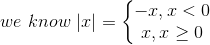Now we have,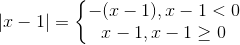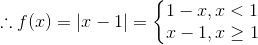Hence, f(x) is defined for all real numbers x.

Domain (f) = R

When, x < 1, we have x – 1 < 0 or 1 – x > 0.

|x – 1| > 0 ⇒ f(x) > 0

When, x ≥ 1, we have x – 1 ≥ 0.

|x – 1| ≥ 0 ⇒ f(x) ≥ 0

∴ f(x) ≥ 0 or f(x) ∈ [0, ∞)

Range (f) = [0, ∞)

(vii) f (x) = -|x|Now we have,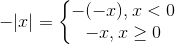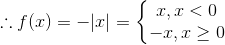Hence, f(x) is defined for all real numbers x.

Domain (f) = R

When, x < 0, we have –|x| < 0

f (x) < 0

When, x ≥ 0, we have –x ≤ 0.

–|x| ≤ 0 ⇒ f (x) ≤ 0

∴ f (x) ≤ 0 or f (x) ∈ (–∞, 0]

Range (f) = (–∞, 0]

(viii) f (x) = √(9-x2)

We know the square of a real number is never negative.

f(x) takes real values only when 9 – x2 ≥ 0

9 ≥ x2

x2 ≤ 9

x2 – 9 ≤ 0

x2 – 32 ≤ 0

(x + 3)(x – 3) ≤ 0

x ≥ –3 and x ≤ 3

∴ x ∈ [–3, 3]

Domain (f) = [–3, 3]

When, x ∈ [–3, 3], we have 0 ≤ 9 – x2 ≤ 9

0 ≤ √(9-x2) ≤ 3 ⇒ 0 ≤ f (x) ≤ 3

∴ f(x) ∈ [0, 3]

Range (f) = [0, 3]

EXERCISE 3.4 PAGE NO: 3.38

1. Find f + g, f – g, cf (c ∈ R, c ≠ 0), fg, 1/f and f/g in each of the following:
(i) f (x) = x3 + 1 and g (x) = x + 1

(ii) f (x) = √(x-1) and g (x) = √(x+1)

Solution:

(i) f (x) = x3 + 1 and g(x) = x + 1

We have f(x): R → R and g(x): R → R

(a) f + g

We know, (f + g) (x) = f(x) + g(x)

(f + g) (x) = x3 + 1 + x + 1

= x3 + x + 2

So, (f + g) (x): R → R

∴ f + g: R → R is given by (f + g) (x) = x3 + x + 2

(b) f – g

We know, (f – g) (x) = f(x) – g(x)

(f – g) (x) = x3 + 1 – (x + 1)

= x3 + 1 – x – 1

= x3 – x

So, (f – g) (x): R → R

∴ f – g: R → R is given by (f – g) (x) = x3 – x

(c) cf (c ∈ R, c ≠ 0)

We know, (cf) (x) = c × f(x)

(cf)(x) = c(x3 + 1)

= cx3 + c

So, (cf) (x) : R → R

∴ cf: R → R is given by (cf) (x) = cx3 + c

(d) fg

We know, (fg) (x) = f(x) g(x)

(fg) (x) = (x3 + 1) (x + 1)

= (x + 1) (x2 – x + 1) (x + 1)

= (x + 1)2 (x2 – x + 1)

So, (fg) (x): R → R

∴ fg: R → R is given by (fg) (x) = (x + 1)2(x2 – x + 1)

(e) 1/f

We know, (1/f) (x) = 1/f (x)

1/f (x) = 1 / (x3 + 1)

Observe that 1/f(x) is undefined when f(x) = 0 or when x = – 1.

So, 1/f: R – {–1} → R is given by 1/f (x) = 1 / (x3 + 1)

(f) f/g

We know, (f/g) (x) = f(x)/g(x)

(f/g) (x) = (x3 + 1) / (x + 1)

Observe that (x3 + 1) / (x + 1) is undefined when g(x) = 0 or when x = –1.

Using x3 + 1 = (x + 1) (x2 – x + 1), we have

(f/g) (x) = [(x+1) (x2– x+1)/(x+1)]

= x2 – x + 1

∴ f/g: R – {–1} → R is given by (f/g) (x) = x2 – x + 1

(ii) f (x) = √(x-1) and g (x) = √(x+1)

We have f(x): [1, ∞) → R+ and g(x): [–1, ∞) → R+ as real square root is defined only for non-negative numbers.

(a) f + g

We know, (f + g) (x) = f(x) + g(x)

(f+g) (x) = √(x-1) + √(x+1)

Domain of (f + g) = Domain of f ∩ Domain of g

Domain of (f + g) = [1, ∞) ∩ [–1, ∞)

Domain of (f + g) = [1, ∞)

∴ f + g: [1, ∞) → R is given by (f+g) (x) = √(x-1) + √(x+1)

(b) f – g

We know, (f – g) (x) = f(x) – g(x)

(f-g) (x) = √(x-1) – √(x+1)

Domain of (f – g) = Domain of f ∩ Domain of g

Domain of (f – g) = [1, ∞) ∩ [–1, ∞)

Domain of (f – g) = [1, ∞)

∴ f – g: [1, ∞) → R is given by (f-g) (x) = √(x-1) – √(x+1)

(c) cf (c ∈ R, c ≠ 0)

We know, (cf) (x) = c × f(x)

(cf) (x) = c√(x-1)

Domain of (cf) = Domain of f

Domain of (cf) = [1, ∞)

∴ cf: [1, ∞) → R is given by (cf) (x) = c√(x-1)

(d) fg

We know, (fg) (x) = f(x) g(x)

(fg) (x) = √(x-1) √(x+1)

= √(x2 -1)

Domain of (fg) = Domain of f ∩ Domain of g

Domain of (fg) = [1, ∞) ∩ [–1, ∞)

Domain of (fg) = [1, ∞)

∴ fg: [1, ∞) → R is given by (fg) (x) = √(x2 -1)

(e) 1/f

We know, (1/f) (x) = 1/f(x)

(1/f) (x) = 1/√(x-1)

Domain of (1/f) = Domain of f

Domain of (1/f) = [1, ∞)

Observe that 1/√(x-1) is also undefined when x – 1 = 0 or x = 1.

∴ 1/f: (1, ∞) → R is given by (1/f) (x) = 1/√(x-1)

(f) f/g

We know, (f/g) (x) = f(x)/g(x)

(f/g) (x) = √(x-1)/√(x+1)

(f/g) (x) = √[(x-1)/(x+1)]

Domain of (f/g) = Domain of f ∩ Domain of g

Domain of (f/g) = [1, ∞) ∩ [–1, ∞)

Domain of (f/g) = [1, ∞)

∴ f/g: [1, ∞) → R is given by (f/g) (x) = √[(x-1)/(x+1)]

2. Let f(x) = 2x + 5 and g(x) = x2 + x. Describe

(i) f + g
(ii) f – g
(iii) fg
(iv) f/g
Find the domain in each case.

Solution:

Given:

f(x) = 2x + 5 and g(x) = x2 + x

Both f(x) and g(x) are defined for all x ∈ R.

So, domain of f = domain of g = R

(i) f + g

We know, (f + g)(x) = f(x) + g(x)

(f + g)(x) = 2x + 5 + x2 + x

= x2 + 3x + 5

(f + g)(x) Is defined for all real numbers x.

∴ The domain of (f + g) is R

(ii) f – g

We know, (f – g)(x) = f(x) – g(x)

(f – g)(x) = 2x + 5 – (x2 + x)

= 2x + 5 – x2 – x

= 5 + x – x2

(f – g)(x) is defined for all real numbers x.

∴ The domain of (f – g) is R

(iii) fg

We know, (fg)(x) = f(x)g(x)

(fg)(x) = (2x + 5)(x2 + x)

= 2x(x2 + x) + 5(x2 + x)

= 2x3 + 2x2 + 5x2 + 5x

= 2x3 + 7x2 + 5x

(fg)(x) is defined for all real numbers x.

∴ The domain of fg is R

(iv) f/g

We know, (f/g) (x) = f(x)/g(x)

(f/g) (x) = (2x+5)/(x2+x)

(f/g) (x) is defined for all real values of x, except for the case when x2 + x = 0.

x2 + x = 0

x(x + 1) = 0

x = 0 or x + 1 = 0

x = 0 or –1

When x = 0 or –1, (f/g) (x) will be undefined as the division result will be indeterminate.

∴ The domain of f/g = R – {–1, 0}

3. If f(x) be defined on [–2, 2] and is given by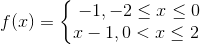and g(x) = f(|x|) + |f(x)|. Find g(x).

Solution:

Given: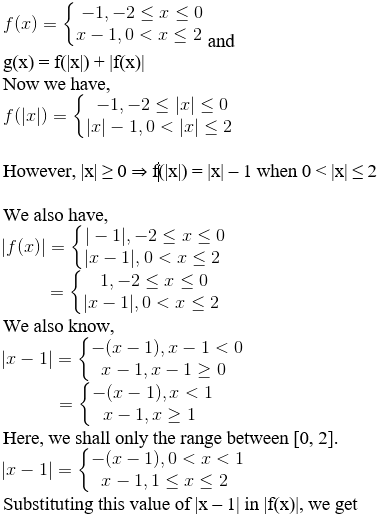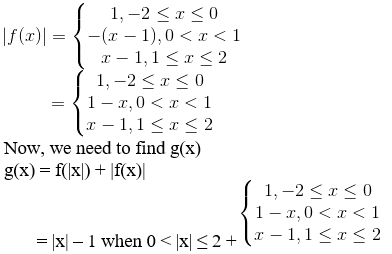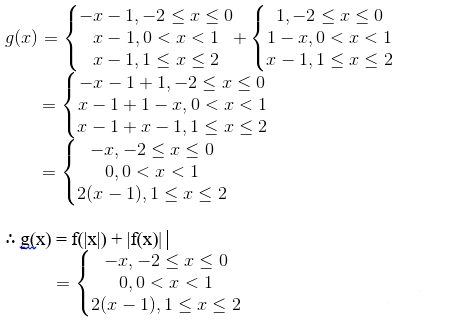4. Let f, g be two real functions defined by f(x) = √(x+1) and g(x) = √(9-x2). Then, describe each of the following functions.
(i) f + g
(ii) g – f
(iii) fg
(iv) f/g
(v) g/f
(vi) 2f – √5g
(vii) f2 + 7f
(viii) 5/g

Solution:

Given:

f(x) = √(x+1) and g(x) = √(9-x2)

We know the square of a real number is never negative.

So, f(x) takes real values only when x + 1 ≥ 0

x ≥ –1, x ∈ [–1, ∞)

Domain of f = [–1, ∞)

Similarly, g(x) takes real values only when 9 – x2 ≥ 0

9 ≥ x2

x2 ≤ 9

x2 – 9 ≤ 0

x2 – 32 ≤ 0

(x + 3)(x – 3) ≤ 0

x ≥ –3 and x ≤ 3

∴ x ∈ [–3, 3]

Domain of g = [–3, 3]

(i) f + g

We know, (f + g)(x) = f(x) + g(x)

(f + g) (x) = √(x+1) + √(9-x2)

Domain of f + g = Domain of f ∩ Domain of g

= [–1, ∞) ∩ [–3, 3]

= [–1, 3]

∴ f + g: [–1, 3] → R is given by (f + g) (x) = f(x) + g(x) = √(x+1) + √(9-x2)

(ii) g – f

We know, (g – f)(x) = g(x) – f(x)

(g – f) (x) = √(9-x2) – √(x+1)

Domain of g – f = Domain of g ∩ Domain of f

= [–3, 3] ∩ [–1, ∞)

= [–1, 3]

∴ g – f: [–1, 3] → R is given by (g – f) (x) = g(x) – f(x) = √(9-x2) – √(x+1)

(iii) fg

We know, (fg) (x) = f(x)g(x)

(fg) (x) = √(x+1) √(9-x2)

= √[(x+1) (9-x2)]

= √[x(9-x2) + (9-x2)]

= √(9x-x3+9-x2)

= √(9+9x-x2-x3)

Domain of fg = Domain of f ∩ Domain of g

= [–1, ∞) ∩ [–3, 3]

= [–1, 3]

∴ fg: [–1, 3] → R is given by (fg) (x) = f(x) g(x) = √(x+1) √(9-x2) = √(9+9x-x2-x3)

(iv) f/g

We know, (f/g) (x) = f(x)/g(x)

(f/g) (x) = √(x+1) / √(9-x2)

= √[(x+1) / (9-x2)]

Domain of f/g = Domain of f ∩ Domain of g

= [–1, ∞) ∩ [–3, 3]

= [–1, 3]

However, (f/g) (x) is defined for all real values of x ∈ [–1, 3], except for the case when 9 – x2 = 0 or x = ± 3

When x = ±3, (f/g) (x) will be undefined as the division result will be indeterminate.

Domain of f/g = [–1, 3] – {–3, 3}

Domain of f/g = [–1, 3)

∴ f/g: [–1, 3) → R is given by (f/g) (x) = f(x)/g(x) = √(x+1) / √(9-x2)

(v) g/f

We know, (g/f) (x) = g(x)/f(x)

(g/f) (x) = √(9-x2) / √(x+1)

= √[(9-x2) / (x+1)]

Domain of g/f = Domain of f ∩ Domain of g

= [–1, ∞) ∩ [–3, 3]

= [–1, 3]

However, (g/f) (x) is defined for all real values of x ∈ [–1, 3], except for the case when x + 1 = 0 or x = –1

When x = –1, (g/f) (x) will be undefined as the division result will be indeterminate.

Domain of g/f = [–1, 3] – {–1}

Domain of g/f = (–1, 3]

∴ g/f: (–1, 3] → R is given by (g/f) (x) = g(x)/f(x) = √(9-x2) / √(x+1)

(vi) 2f – √5g

We know, (2f – √5g) (x) = 2f(x) – √5g(x)

(2f – √5g) (x) = 2f (x) – √5g (x)

= 2√(x+1) – √5√(9-x2)

= 2√(x+1) – √(45- 5x2)

Domain of 2f – √5g = Domain of f ∩ Domain of g

= [–1, ∞) ∩ [–3, 3]

= [–1, 3]

∴ 2f – √5g: [–1, 3] → R is given by (2f – √5g) (x) = 2f (x) – √5g (x) = 2√(x+1) – √(45- 5x2)

(vii) f2 + 7f

We know, (f2 + 7f) (x) = f2(x) + (7f)(x)

(f2 + 7f) (x) = f(x) f(x) + 7f(x)

= √(x+1) √(x+1) + 7√(x+1)

= x + 1 + 7√(x+1)

Domain of f2 + 7f is same as domain of f.

Domain of f2 + 7f = [–1, ∞)

∴ f2 + 7f: [–1, ∞) → R is given by (f2 + 7f) (x) = f(x) f(x) + 7f(x) = x + 1 + 7√(x+1)

(viii) 5/g

We know, (5/g) (x) = 5/g(x)

(5/g) (x) = 5/√(9-x2)

Domain of 5/g = Domain of g = [–3, 3]

However, (5/g) (x) is defined for all real values of x ∈ [–3, 3], except for the case when 9 – x2 = 0 or x = ± 3

When x = ±3, (5/g) (x) will be undefined as the division result will be indeterminate.

Domain of 5/g = [–3, 3] – {–3, 3}

= (–3, 3)

∴ 5/g: (–3, 3) → R is given by (5/g) (x) = 5/g(x) = 5/√(9-x2)

5. If f(x) = loge (1 – x) and g(x) = [x], then determine each of the following functions:
(i) f + g
(ii) fg

(iii) f/g
(iv) g/f

Also, find (f + g) (–1), (fg) (0), (f/g) (1/2) and (g/f) (1/2).

Solution:

Given:

f(x) = loge (1 – x) and g(x) = [x]

We know, f(x) takes real values only when 1 – x > 0

1 > x

x < 1, ∴ x ∈ (–∞, 1)

Domain of f = (–∞, 1)

Similarly, g(x) is defined for all real numbers x.

Domain of g = [x], x ∈ R

= R

(i) f + g

We know, (f + g) (x) = f(x) + g(x)

(f + g) (x) = loge (1 – x) + [x]

Domain of f + g = Domain of f ∩ Domain of g

Domain of f + g = (–∞, 1) ∩ R

= (–∞, 1)

∴ f + g: (–∞, 1) → R is given by (f + g) (x) = loge (1 – x) + [x]

(ii) fg

We know, (fg) (x) = f(x) g(x)

(fg) (x) = loge (1 – x) × [x]

= [x] loge (1 – x)

Domain of fg = Domain of f ∩ Domain of g

= (–∞, 1) ∩ R

= (–∞, 1)

∴ fg: (–∞, 1) → R is given by (fg) (x) = [x] loge (1 – x)

(iii) f/g

We know, (f/g) (x) = f(x)/g(x)

(f/g) (x) = loge (1 – x) / [x]

Domain of f/g = Domain of f ∩ Domain of g

= (–∞, 1) ∩ R

= (–∞, 1)

However, (f/g) (x) is defined for all real values of x ∈ (–∞, 1), except for the case when [x] = 0.

We have, [x] = 0 when 0 ≤ x < 1 or x ∈ [0, 1)

When 0 ≤ x < 1, (f/g) (x) will be undefined as the division result will be indeterminate.

Domain of f/g = (–∞, 1) – [0, 1)

= (–∞, 0)

∴ f/g: (–∞, 0) → R is given by (f/g) (x) = loge (1 – x) / [x]

(iv) g/f

We know, (g/f) (x) = g(x)/f(x)

(g/f) (x) = [x] / loge (1 – x)

However, (g/f) (x) is defined for all real values of x ∈ (–∞, 1), except for the case when loge (1 – x) = 0.

loge (1 – x) = 0 ⇒ 1 – x = 1 or x = 0

When x = 0, (g/f) (x) will be undefined as the division result will be indeterminate.

Domain of g/f = (–∞, 1) – {0}

= (–∞, 0) ∪ (0, 1)

∴ g/f: (–∞, 0) ∪ (0, 1) → R is given by (g/f) (x) = [x] / loge (1 – x)

(a) We need to find (f + g) (–1).

We have, (f + g) (x) = loge (1 – x) + [x], x ∈ (–∞, 1)

Substituting x = –1 in the above equation, we get

(f + g)(–1) = loge (1 – (–1)) + [–1]

= loge (1 + 1) + (–1)

= loge2 – 1

∴ (f + g) (–1) = loge2 – 1

(b) We need to find (fg) (0).

We have, (fg) (x) = [x] loge (1 – x), x ∈ (–∞, 1)

Substituting x = 0 in the above equation, we get

(fg) (0) =  loge (1 – 0)

= 0 × loge1

∴ (fg) (0) = 0

(c) We need to find (f/g) (1/2)

We have, (f/g) (x) = loge (1 – x) / [x], x ∈ (–∞, 0)

However, 1/2 is not in the domain of f/g.

∴ (f/g) (1/2) does not exist.

(d) We need to find (g/f) (1/2)

We have, (g/f) (x) = [x] / loge (1 – x), x ∈ (–∞, 0) ∪ (0, ∞)

Substituting x=1/2 in the above equation, we get

(g/f) (1/2) = [x] / loge (1 – x)

= (1/2)/ loge (1 – 1/2)

= 0.5/ loge (1/2)

= 0 / loge (1/2)

= 0

∴ (g/f) (1/2) = 0

## Frequently Asked Questions on RD Sharma Solutions for Class 11 Maths Chapter 3

### Is BYJU’S website providing answers for RD Sharma Solutions for Class 11 Maths Chapter 3 Functions?

Yes, you can avail the PDFs of RD Sharma Solutions for Class 11 Maths Chapter 3. These solutions are formulated by expert faculty at BYJU’S in an unique way. Also they provide solutions for Class 1 to 12 RD Sharma Textbooks in free PDFs. Who wish to score high in exams are advised to solve the RD Sharma Textbook.

### Mention the important concepts that you learn in RD Sharma Solutions for Class 11 Maths Chapter 3 Functions?

The concepts presented in RD Sharma Solutions for Class 11 Maths Chapter 3 are listed here:
Function as a special kind of relation.
Function as a correspondence.
Description of a function.
Domain, co-domain and range of a function.
Equal functions.
Real functions.
The domain of real functions.
Range of real functions.
Some standard real functions and their graphs.
Operations on real functions.

### What is the meaning of function according to RD Sharma Solutions for Class 11 Maths Chapter 3?

According to RD Sharma Solutions for Class 11 Maths Chapter 3 a function is a relation between a set of inputs and a set of permissible outputs with the property that each input is related to exactly one output. Let A & B be any two non-empty sets, mapping from A to B will be a function only when every element in set A has one end only one image in set B.